Everyone is welcome here --- except those who have borrowed books from me for and have not returned them yet!

# Examples of basic data analyses with R

Posted on décembre 19, 2015 in stats

# Estimating a proportion from a sample

Here, we show how to get a confidence interval for the proportion of the mean obtained from a sample.

Let us generate 100 random flips of a biased coin (p=1/3)

n <- 100
a <- rbinom(n, size=1, prob=1/3)

To obtain descriptive statistics, one can use the functions table or prop.table:

table(a)
## a
##  0  1
## 61 39
prop.table(table(a))
## a
##    0    1
## 0.61 0.39
mean(a)
##  0.39

Note the existence of two other functions that also tabulate the content of discrete variable(s) — ftable and xtabs — and offer more flexibility to display the output. The latter, xtab is particularily powerful for crosstabulating several variables.

You can plot the distributions of values using stick plots or barplots.

plot(table(a))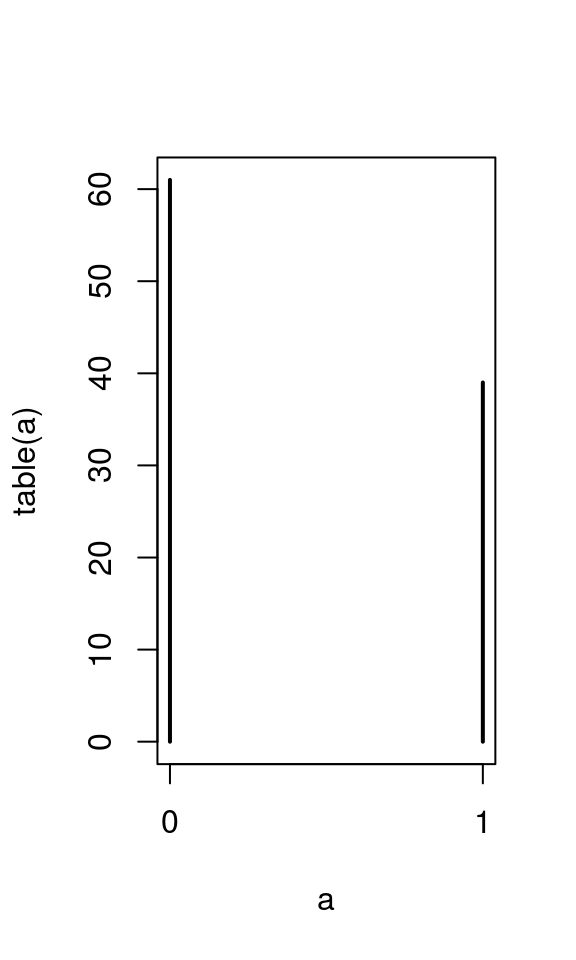barplot(table(a))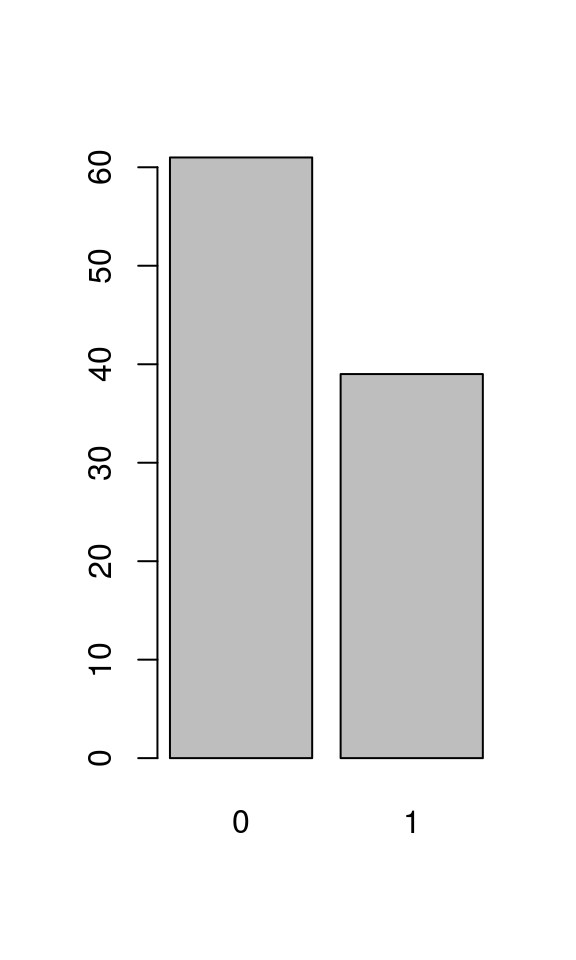The relevant function to test against the null hypothesis of p=0.5 and to obtain a confidence interval is prop.test:

prop.test(table(a))
##
##  1-sample proportions test with continuity correction
##
## data:  table(a), null probability 0.5
## X-squared = 4.41, df = 1, p-value = 0.03573
## alternative hypothesis: true p is not equal to 0.5
## 95 percent confidence interval:
##  0.5070114 0.7044326
## sample estimates:
##    p
## 0.61
prop.test(table(a))$conf.int ##  0.5070114 0.7044326 ## attr(,"conf.level") ##  0.95 ## Comparing two empirical proportions Suppose we have two binomial samples. To test wehterh the underlyinh probability is the same, we enter the counts in a 2x2 matrix where each column gives the counts of successes and failures and use prop.test again: twoprop <- matrix(c(105, 366, 45, 67), nrow=2) prop.test(twoprop) ## ## 2-sample test for equality of proportions with continuity ## correction ## ## data: twoprop ## X-squared = 14.226, df = 1, p-value = 0.0001621 ## alternative hypothesis: two.sided ## 95 percent confidence interval: ## -0.23061397 -0.05991721 ## sample estimates: ## prop 1 prop 2 ## 0.7000000 0.8452656 See also fisher.test. # Estimating the mean of a population from a sample Let us now consider the case of a continuous variable. n <- 100 a <- rnorm(n, mean=100, sd=15) ## Exploratory graphics There are several ways to graphically inspect a continous variable. Which one is approriate depends on the number of data points and their distribution. par(las=1) stripchart(a, method='jitter', vertical=TRUE) abline(h=mean(a), lty=2)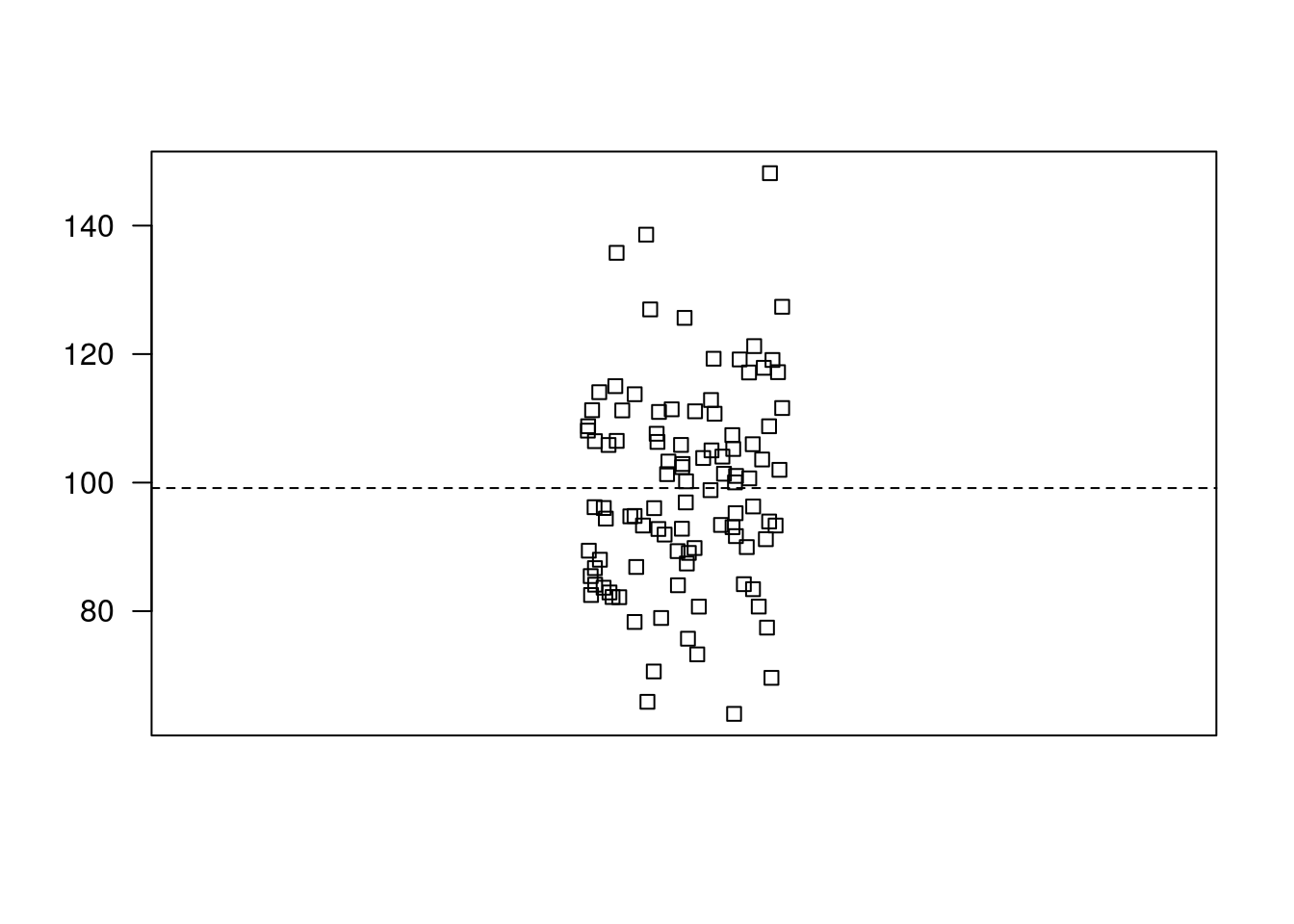If the sample is small, I recommend using a dotchart: dotchart(a[1:20])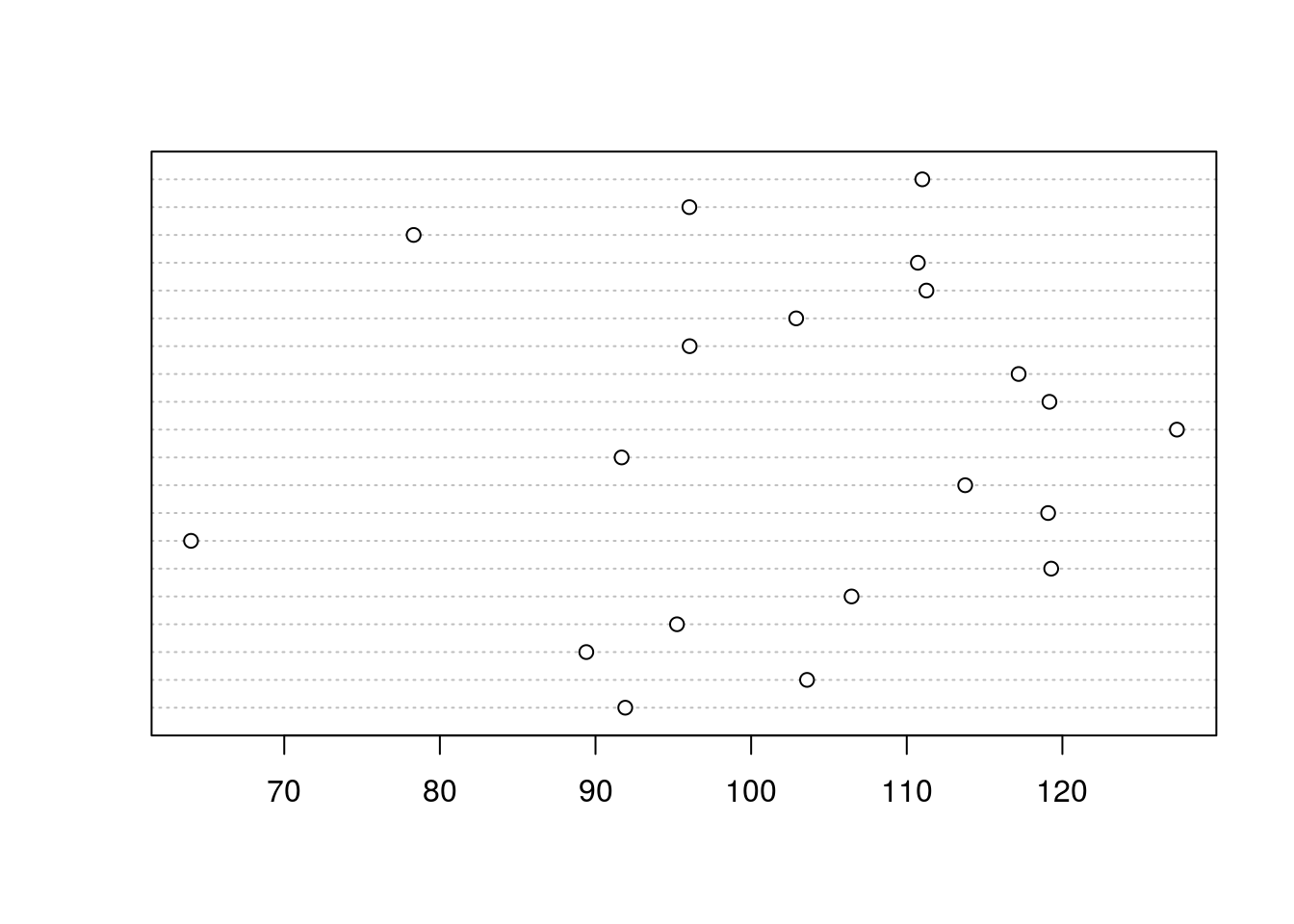If the sample is large enough, histograms or density plots are a good idea: hist(a) rug(a)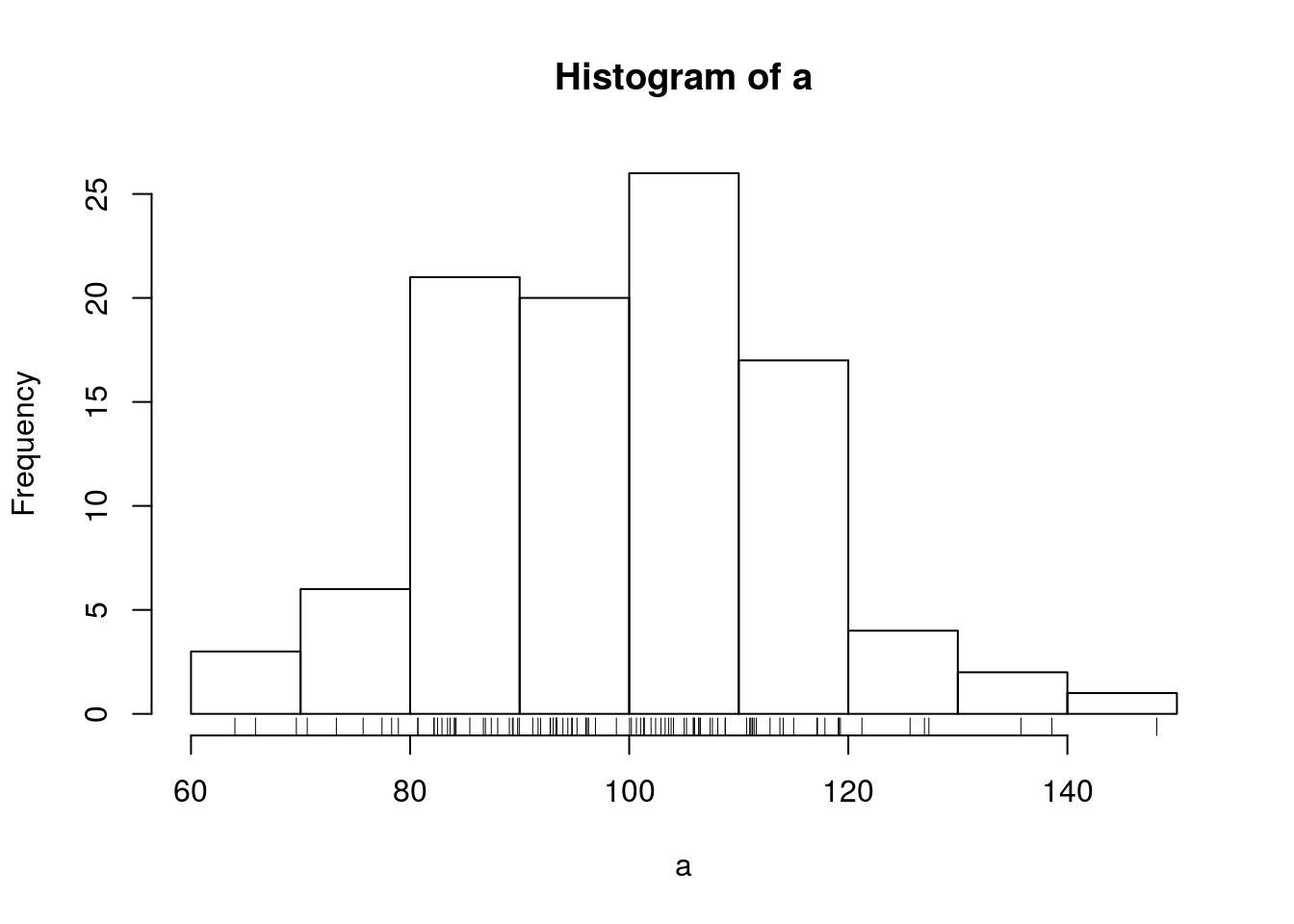plot(density(a)) abline(v=mean(a), lty=2) rug(a)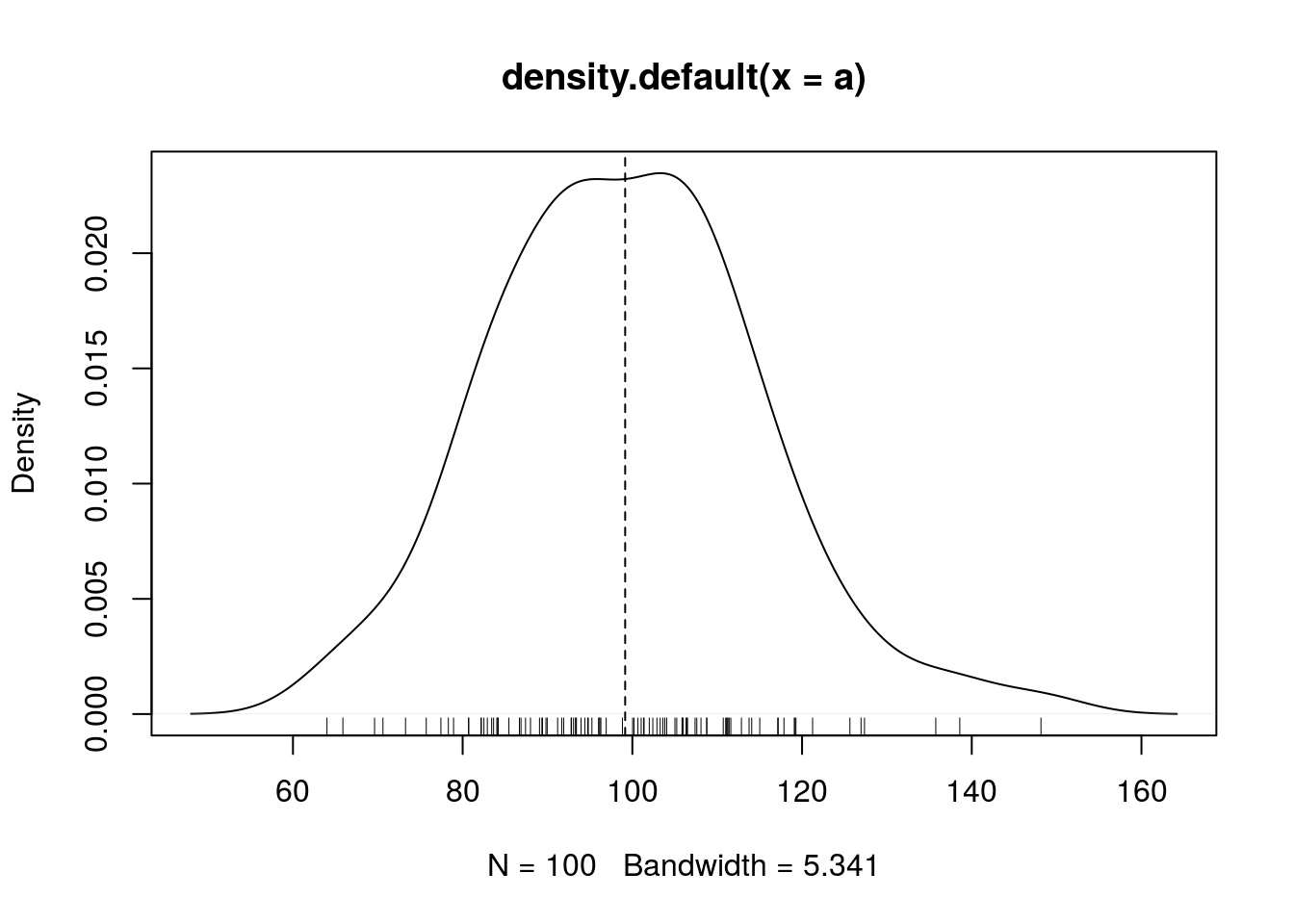boxplot(a)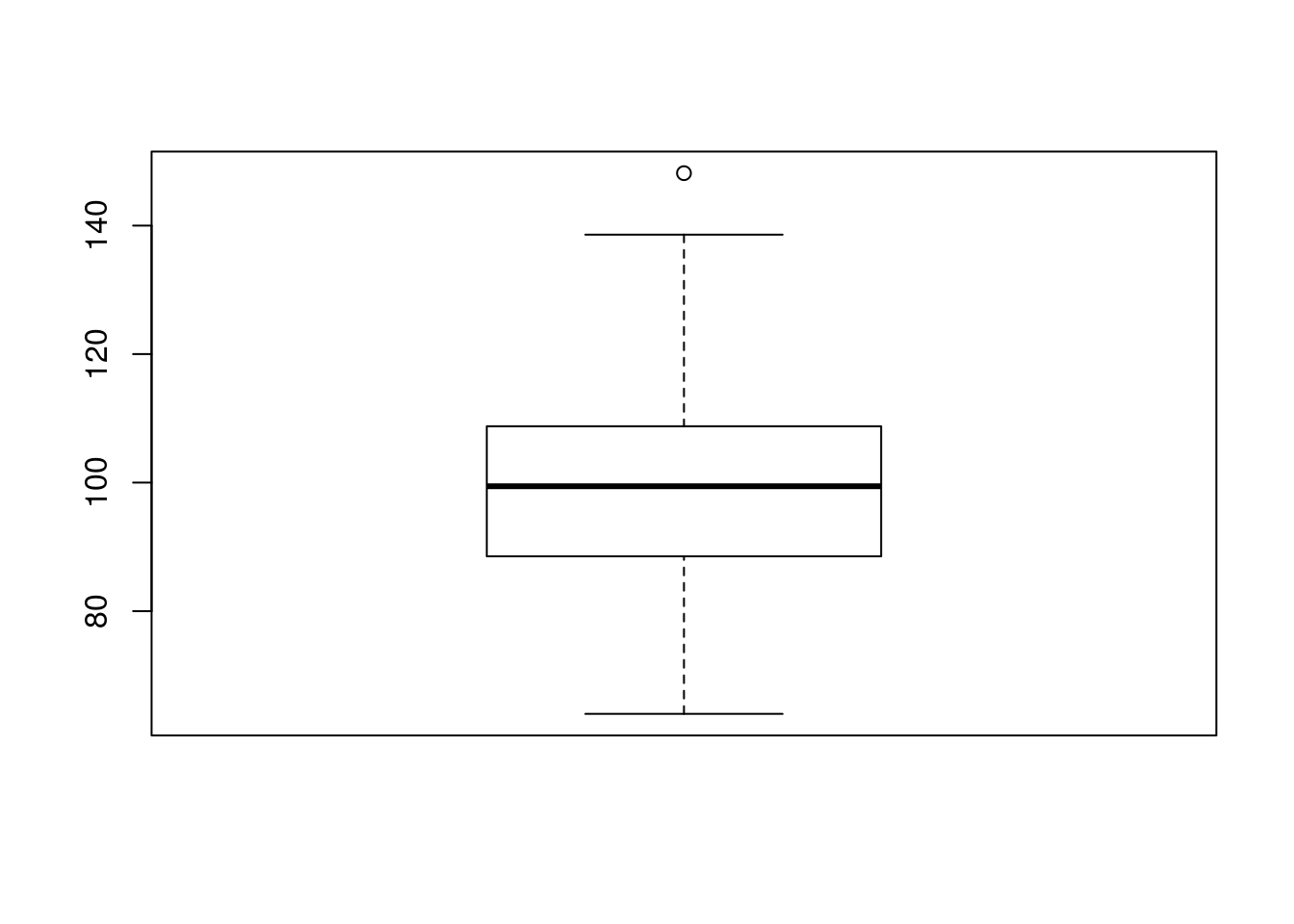## Descriptive statistics summary(a) ## Min. 1st Qu. Median Mean 3rd Qu. Max. ## 64.01 88.79 99.43 99.15 108.80 148.20 mean(a) ##  99.15297 mean(a[abs(a-mean(a)) < 2*sd(a)]) # after deleting data points beyond 2 standard deviations  ##  98.5568 ## Confidence interval of a mean Assuming normally distributed values: t.test(a) ## ## One Sample t-test ## ## data: a ## t = 63.245, df = 99, p-value < 2.2e-16 ## alternative hypothesis: true mean is not equal to 0 ## 95 percent confidence interval: ## 96.04219 102.26375 ## sample estimates: ## mean of x ## 99.15297 t.test(a)$conf.int
##   96.04219 102.26375
## attr(,"conf.level")
##  0.95

A confidence interval based on bootstrap can also be obtained:

require(boot)
## Loading required package: boot
sampmean <- function(x, d) { mean(x[d]) }
boota <- boot(a, sampmean, 1000)
boot.ci(boota)
## Warning in boot.ci(boota): bootstrap variances needed for studentized
## intervals
## BOOTSTRAP CONFIDENCE INTERVAL CALCULATIONS
## Based on 1000 bootstrap replicates
##
## CALL :
## boot.ci(boot.out = boota)
##
## Intervals :
## Level      Normal              Basic
## 95%   ( 96.14, 102.25 )   ( 96.17, 102.20 )
##
## Level     Percentile            BCa
## 95%   ( 96.10, 102.14 )   ( 96.13, 102.15 )
## Calculations and Intervals on Original Scale

# Comparing two groups (continous dependent variable)

g1 <- 500 + rnorm(30, sd=40)
g2 <- 520 + rnorm(20, sd=30)
write(g1, 'group1.dat')
write(g2, 'group2.dat')
rm(g1, g2)

Data for this example are in two text files group1.dat and group2.dat.

g1 <- scan('group1.dat')
g2 <- scan('group2.dat')

We arrange them into a data frame with two columns: group (a factor with two modalities: Gr1 and Gr2), and y which contains the values themselves.

tg <- data.frame(group=factor(rep(c('Gr1', 'Gr2'),
c(length(g1), length(g2)))),
y=c(g1, g2))

head(tg)
##   group        y
## 1   Gr1 545.8721
## 2   Gr1 543.7691
## 3   Gr1 557.8433
## 4   Gr1 483.4303
## 5   Gr1 408.2971
## 6   Gr1 537.6479
str(tg)
## 'data.frame':    50 obs. of  2 variables:
##  $group: Factor w/ 2 levels "Gr1","Gr2": 1 1 1 1 1 1 1 1 1 1 ... ##$ y    : num  546 544 558 483 408 ...
table(tg$group) ## ## Gr1 Gr2 ## 30 20 ### Graphical explorations hist(tg$y)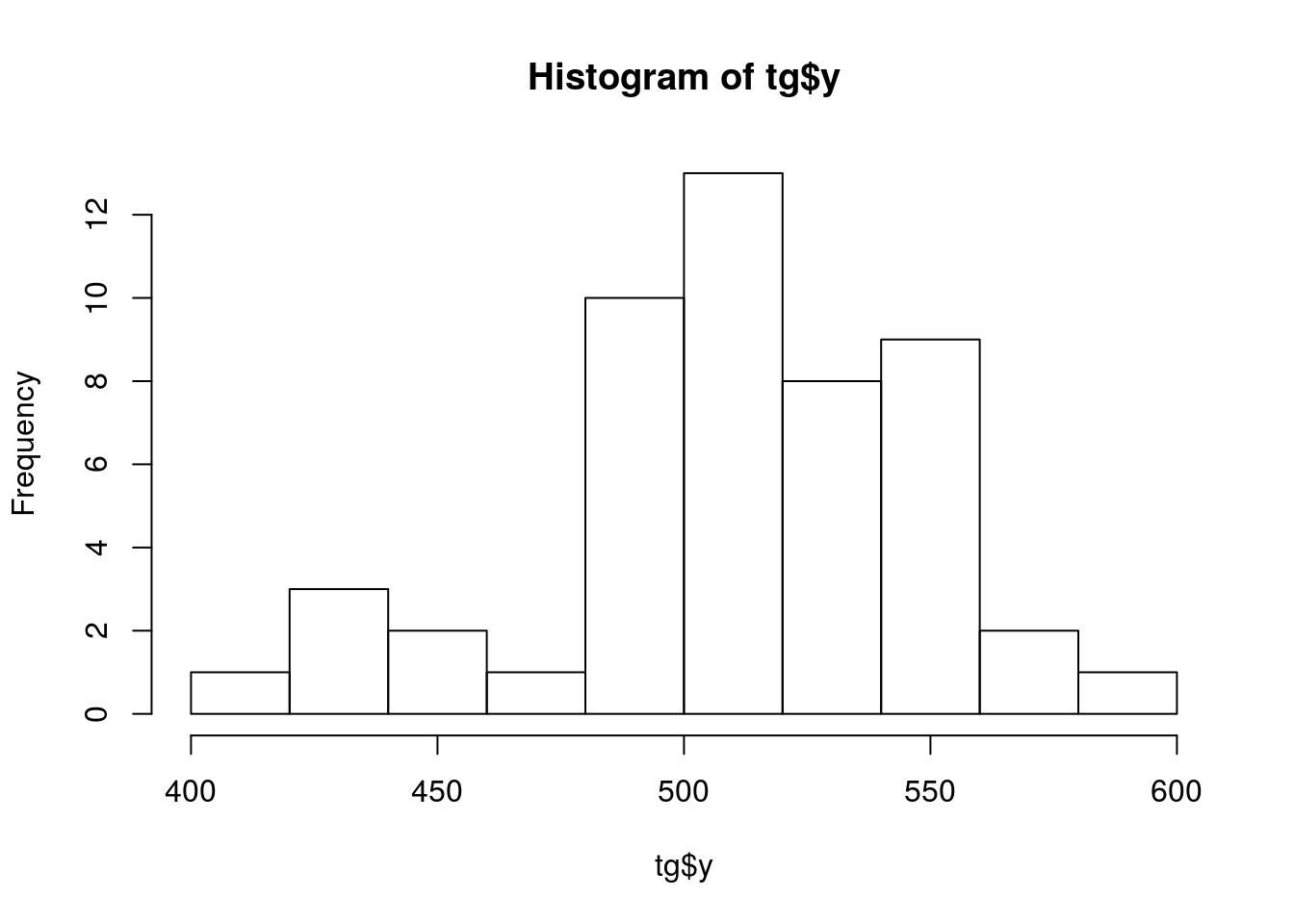boxplot(tg$y ~ tg$group)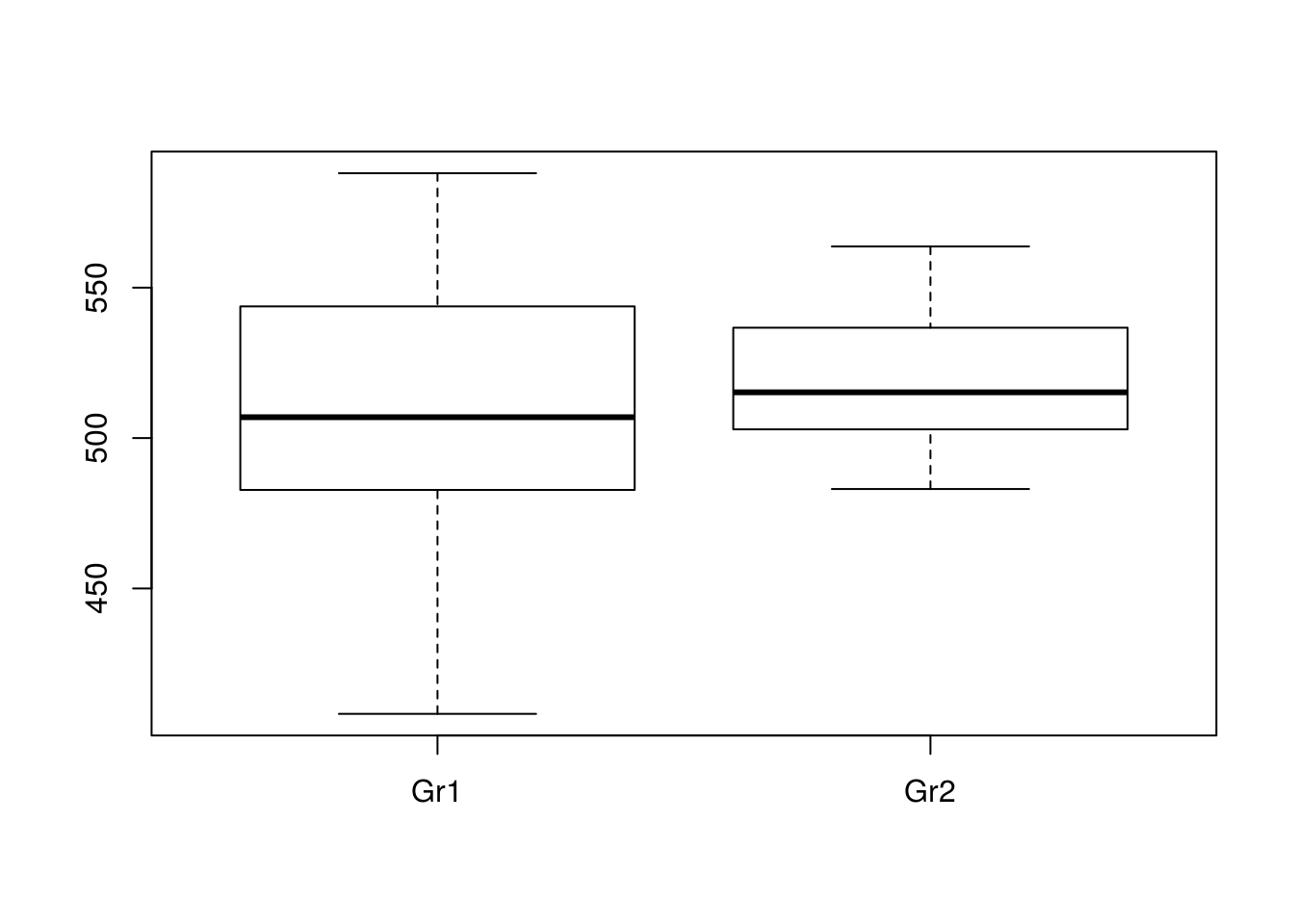require(lattice)
## Loading required package: lattice
##
## Attaching package: 'lattice'
## The following object is masked from 'package:boot':
##
##     melanoma
dotchart(tg$y, groups = tg$group)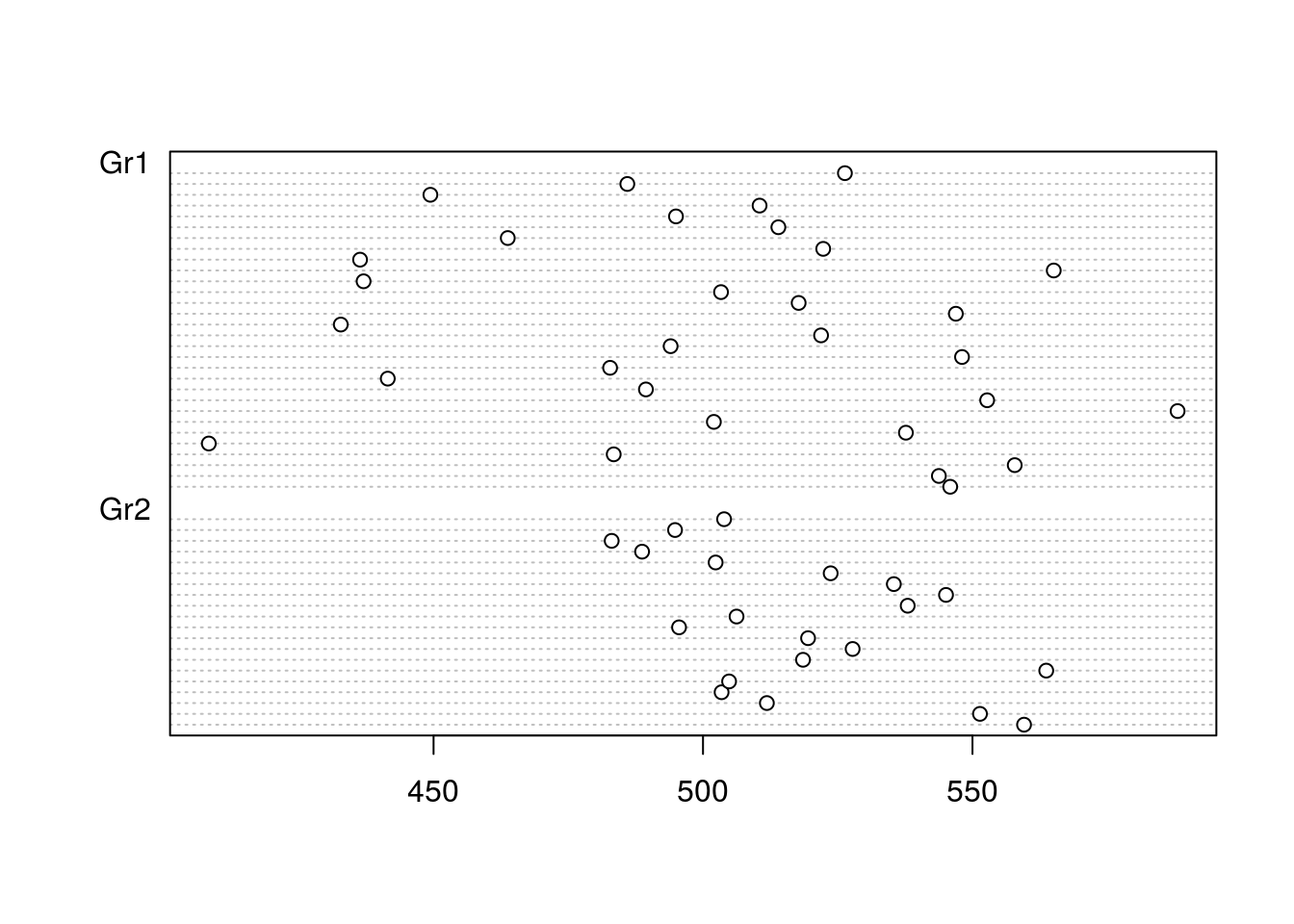When the samples are small, stripchart may be the best:

stripchart(tg$y ~ tg$group,
vertical=TRUE,
pch=1)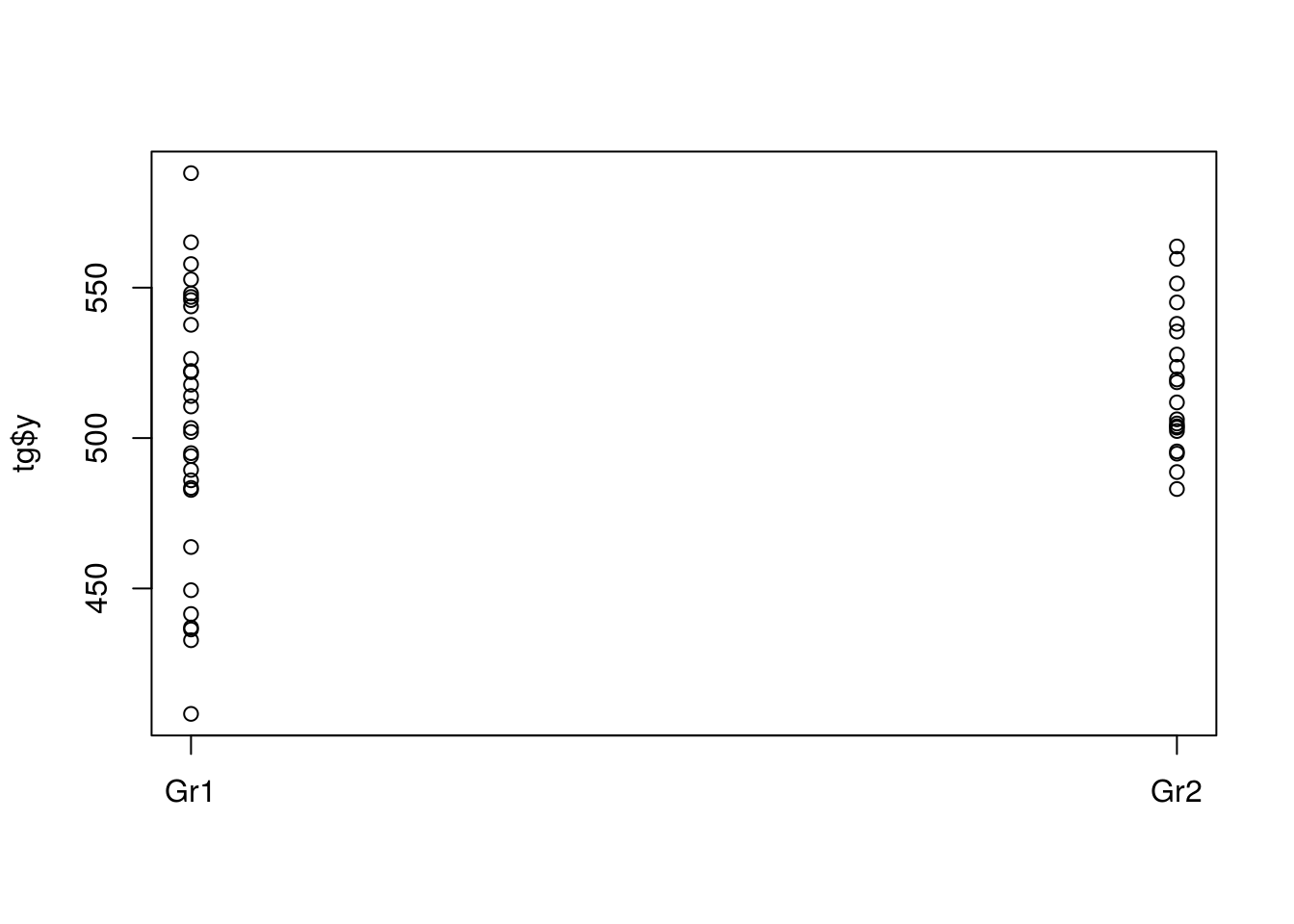If the samples are large enough, you can create density plots:

par(mfrow=(c(1, 2)))
xsca <- range(tg$y) for (gr in levels(tg$group))
{
with(subset(tg, group==gr),
{
plot(density(y), xlim=xsca, main=gr, bty='l')
rug(y, ticksize=0.1)
})
}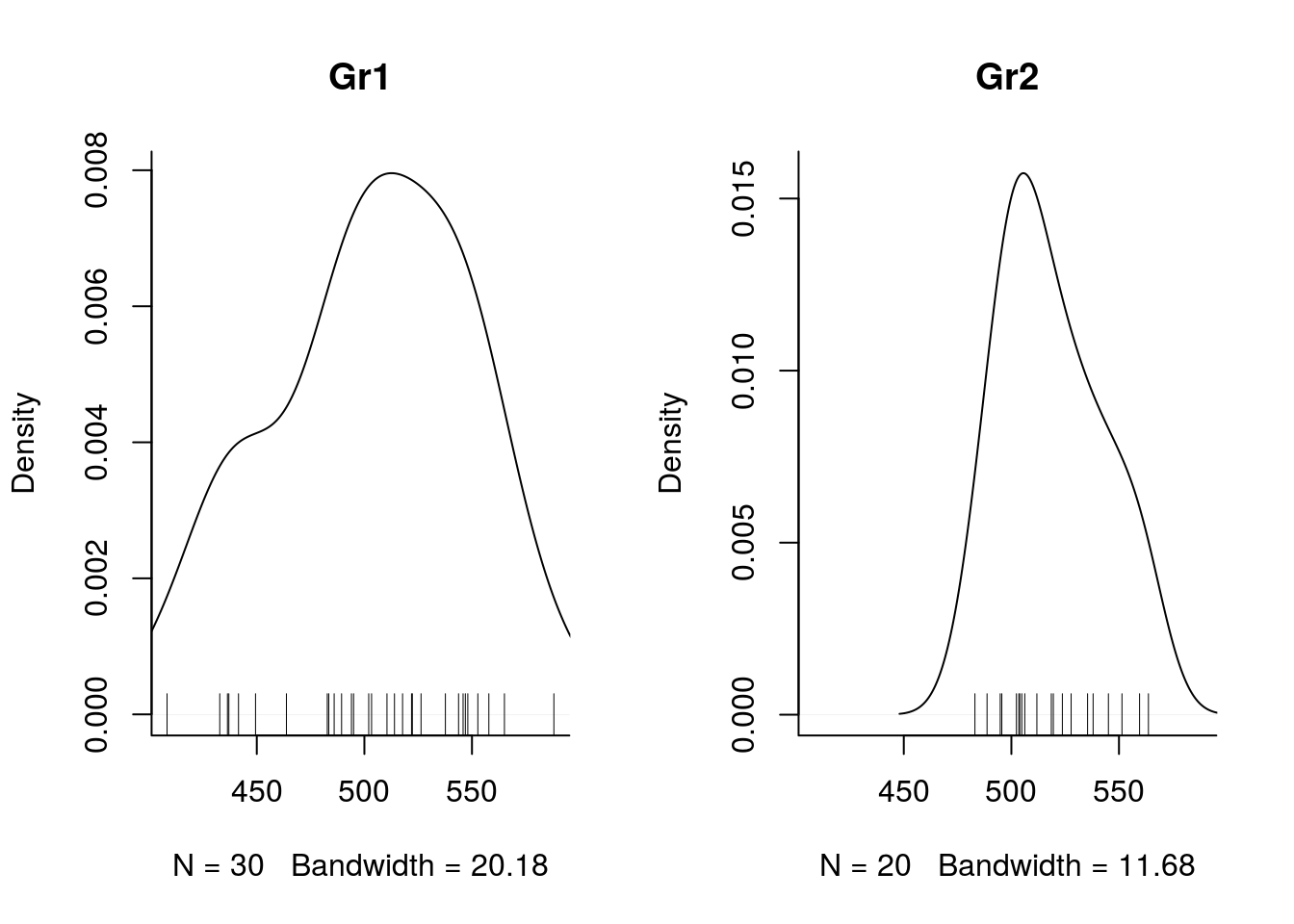Or violin plots:

require(ggplot2)
## Loading required package: ggplot2
p <-  ggplot(tg, aes(group, y))
p + geom_violin() + geom_jitter(height = 0, width = 0.1)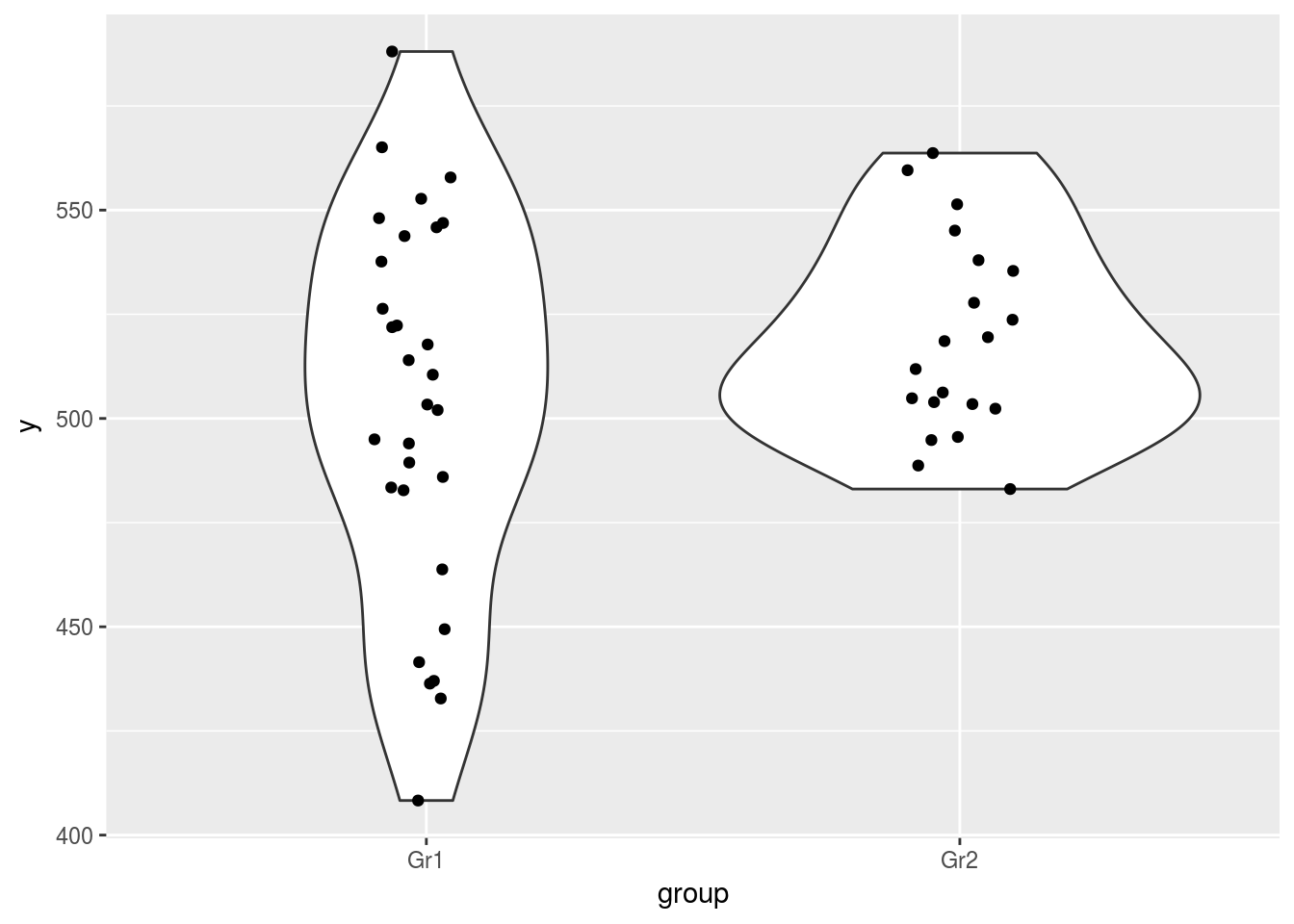To obtain the basic descriptive stats

attach(tg)
signif(tapply(y, group, mean),3)
## Gr1 Gr2
## 503 519
signif(tapply(y, group, median), 3)
## Gr1 Gr2
## 507 515
signif(tapply(y, group, sd), 3)
##  Gr1  Gr2
## 45.2 23.6
signif(tapply(y, group, se), 3)
##  Gr1  Gr2
## 8.25 5.28
detach(tg)

### Inferential statistics

Student T-tests. First assuming equal variance, then relaxing this assumption

t.test(y ~ group, data=tg, var.equal=TRUE)
##
##  Two Sample t-test
##
## data:  y by group
## t = -1.3998, df = 48, p-value = 0.168
## alternative hypothesis: true difference in means is not equal to 0
## 95 percent confidence interval:
##  -37.543155   6.724562
## sample estimates:
## mean in group Gr1 mean in group Gr2
##          503.4598          518.8691
t.test(y ~ group, data=tg)
##
##  Welch Two Sample t-test
##
## data:  y by group
## t = -1.5731, df = 45.889, p-value = 0.1226
## alternative hypothesis: true difference in means is not equal to 0
## 95 percent confidence interval:
##  -35.128392   4.309799
## sample estimates:
## mean in group Gr1 mean in group Gr2
##          503.4598          518.8691

First with a parametrisation (contr.treatment) of group where the intercept will correspond to the mean of group 1 and the effect will estimate the difference between the two groups.

contrasts(tg$group) <- contr.treatment contrasts(tg$group)
##     2
## Gr1 0
## Gr2 1
summary(lm(y ~ group, data=tg))
##
## Call:
## lm(formula = y ~ group, data = tg)
##
## Residuals:
##     Min      1Q  Median      3Q     Max
## -95.163 -19.398  -0.215  25.384  84.606
##
## Coefficients:
##             Estimate Std. Error t value Pr(>|t|)
## (Intercept)  503.460      6.962   72.31   <2e-16 ***
## group2        15.409     11.008    1.40    0.168
## ---
## Signif. codes:  0 '***' 0.001 '**' 0.01 '*' 0.05 '.' 0.1 ' ' 1
##
## Residual standard error: 38.13 on 48 degrees of freedom
## Multiple R-squared:  0.03922,    Adjusted R-squared:  0.0192
## F-statistic: 1.959 on 1 and 48 DF,  p-value: 0.168

Alternatively, one can prefer a parametrisation where the intercept estimates the global mean and the first parameter is the deviation from the global mean.

contrasts(tg$group) <- contr.sum contrasts(tg$group)
##     [,1]
## Gr1    1
## Gr2   -1
summary(lm(y ~ group, data=tg))
##
## Call:
## lm(formula = y ~ group, data = tg)
##
## Residuals:
##     Min      1Q  Median      3Q     Max
## -95.163 -19.398  -0.215  25.384  84.606
##
## Coefficients:
##             Estimate Std. Error t value Pr(>|t|)
## (Intercept)  511.164      5.504   92.87   <2e-16 ***
## group1        -7.705      5.504   -1.40    0.168
## ---
## Signif. codes:  0 '***' 0.001 '**' 0.01 '*' 0.05 '.' 0.1 ' ' 1
##
## Residual standard error: 38.13 on 48 degrees of freedom
## Multiple R-squared:  0.03922,    Adjusted R-squared:  0.0192
## F-statistic: 1.959 on 1 and 48 DF,  p-value: 0.168

### Barplot with standard errors

Barplot with the means and their associated standard errors (note this is not the standard error for the difference between the groups’ means, which is roughly $$\sqrt{2}$$ larger and, maybe for this reason, rarely used in psychology papers (like they rarely report confidence intervals))

attach(tg)
par(mfrow=c(1,1))
means <- tapply(y, group, mean)
ses <- tapply(y, group, se)

ysca = c(min(means - 3 * ses), max(means + 3 * ses))

mp <- barplot(means, ylim=ysca, xpd=F)
arrows(mp, means-ses,
mp, means+ses,
code=3, angle=90)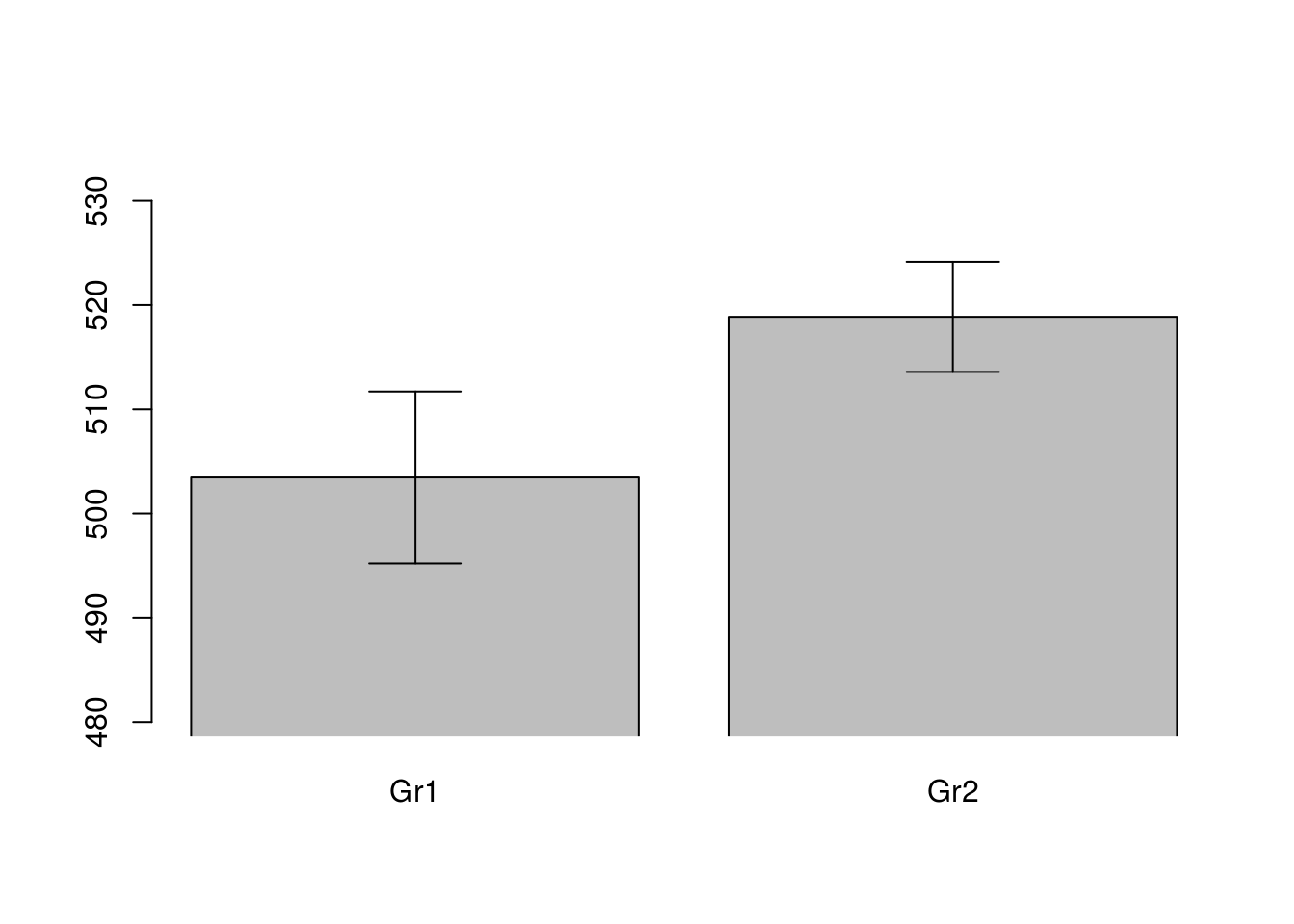detach(tg)

A much nicer plot can be constructed, with confidence intervals for the means and for their difference (Cumming, Geoff, and Sue Finch. 2005. “Inference by Eye: Confidence Intervals and How to Read Pictures of Data.” American Psychologist 60 (2): 170–180.)

attach(tg)
m1 <- t.test(y[group=='Gr1'])$conf.int m2 <- t.test(y[group=='Gr2'])$conf.int
di <- diff(t.test(y~group)$conf.int) ysca <- c(min(c(m1,m2)-0.3*diff(range(c(m1,m2)))), max(c(m1,m2)+0.3*diff(range(c(m1,m2))))) plot(c(Gr1=1, Gr2=2, difference=3), c(mean(m1), mean(m2), mean(m2)), pch=c(16,16,17), ylim=ysca, xlim=c(.5,3.5), axes=F, xlab='', ylab='') axis(2, las=1) axis(1,at=1:3,labels=c('Gr1','Gr2','difference')) arrows(1:3, c(m1, m2, mean(m2)-di/2), 1:3, c(m1, m2, mean(m2)+di/2), code=3, angle=90) abline(h=mean(m1), lty=2) abline(h=mean(m2), lty=2)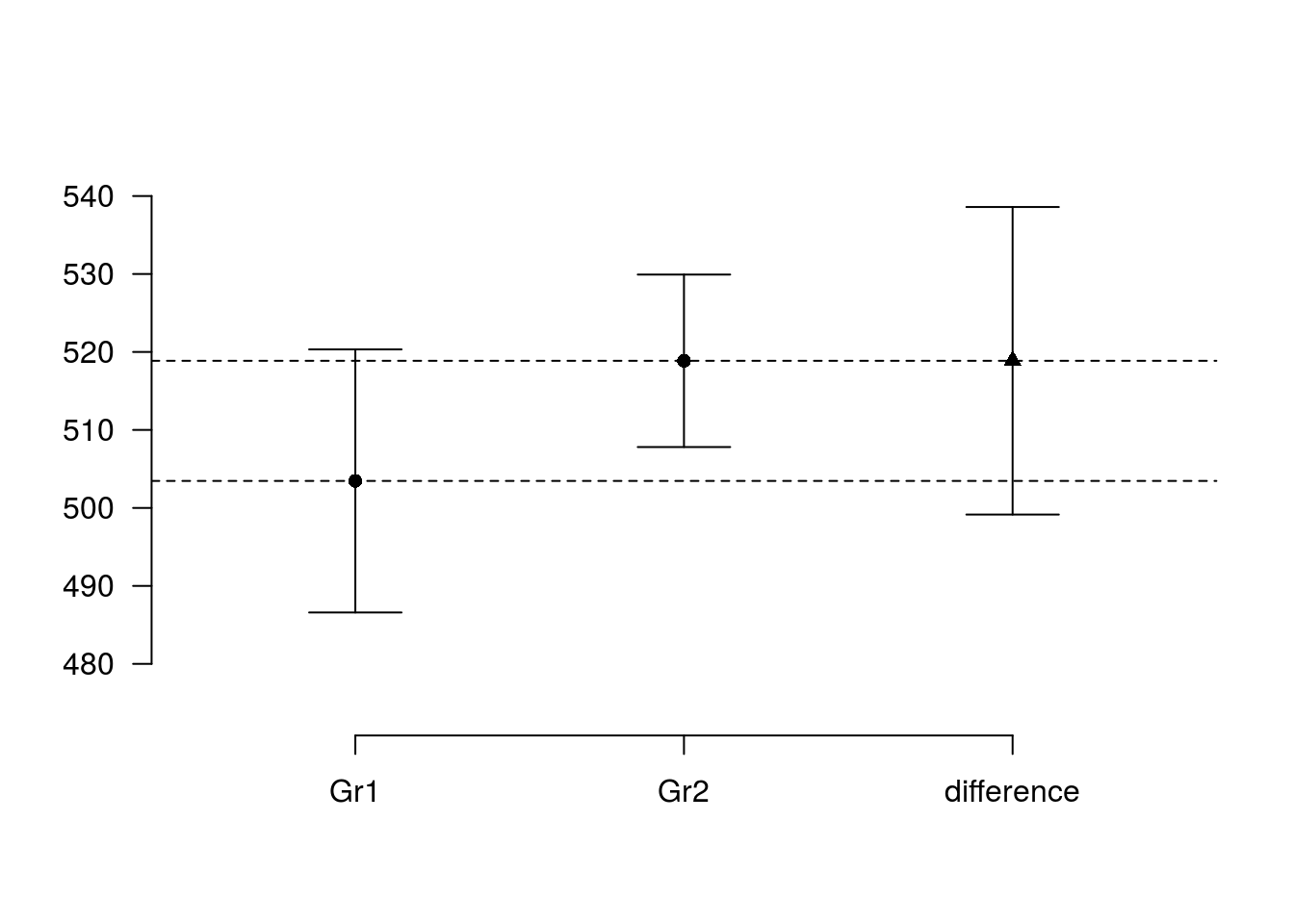detach(tg) # Comparing the means of several independent groups Data are in a spreasheet format, in oneway.csv ow <- read.csv('oneway.csv') head(ow) ## X group y ## 1 1 Gr1 6.697519 ## 2 2 Gr1 5.707240 ## 3 3 Gr1 7.752619 ## 4 4 Gr1 6.451629 ## 5 5 Gr1 5.423243 ## 6 6 Gr1 7.183462 str(ow) ## 'data.frame': 288 obs. of 3 variables: ##$ X    : int  1 2 3 4 5 6 7 8 9 10 ...
##  $group: Factor w/ 5 levels "Gr1","Gr2","Gr3",..: 1 1 1 1 1 1 1 1 1 1 ... ##$ y    : num  6.7 5.71 7.75 6.45 5.42 ...

We can perform the same operations as we did for the two samples case.

### Graphical explorations

attach(ow)
hist(y)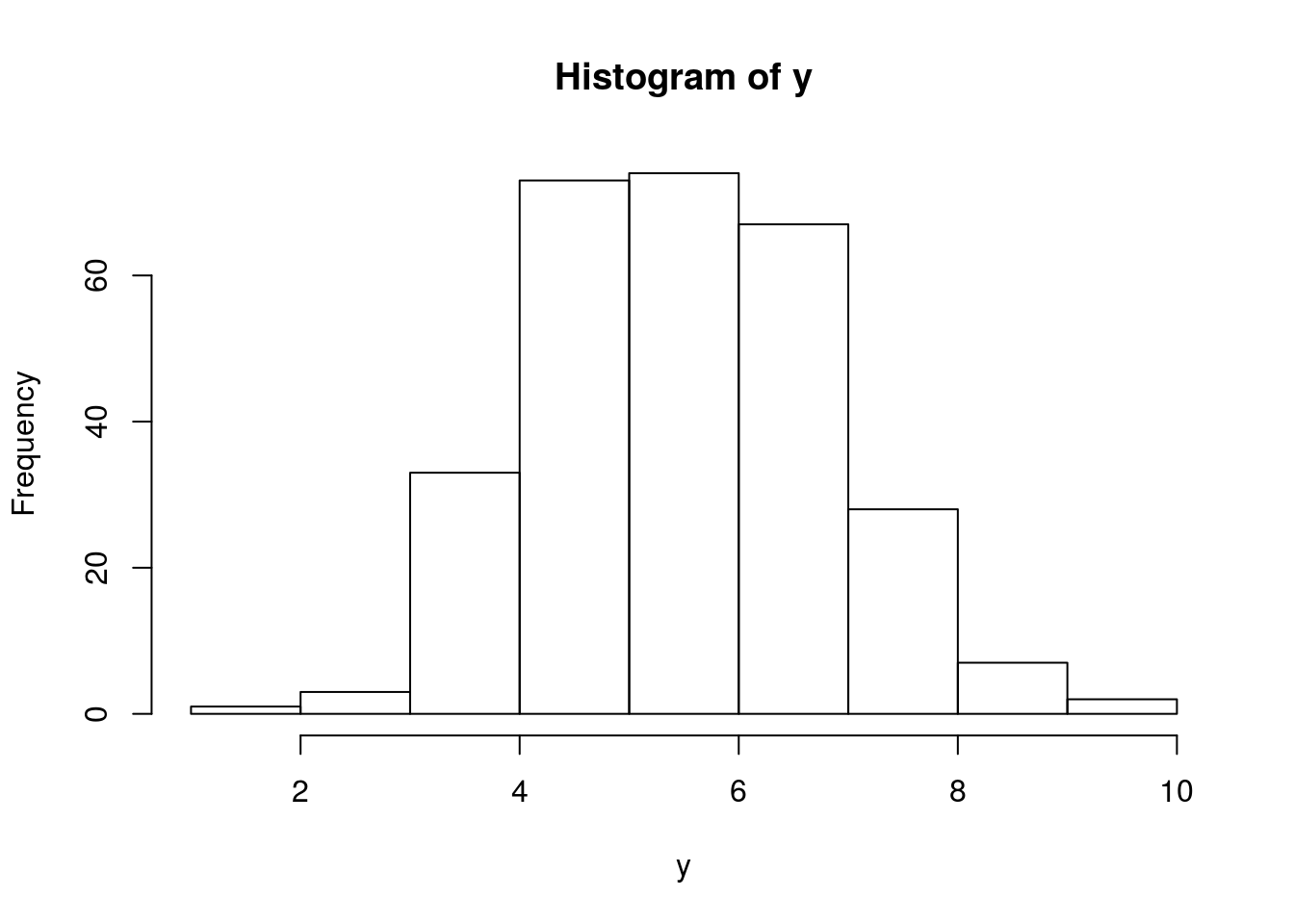plot(y ~ group)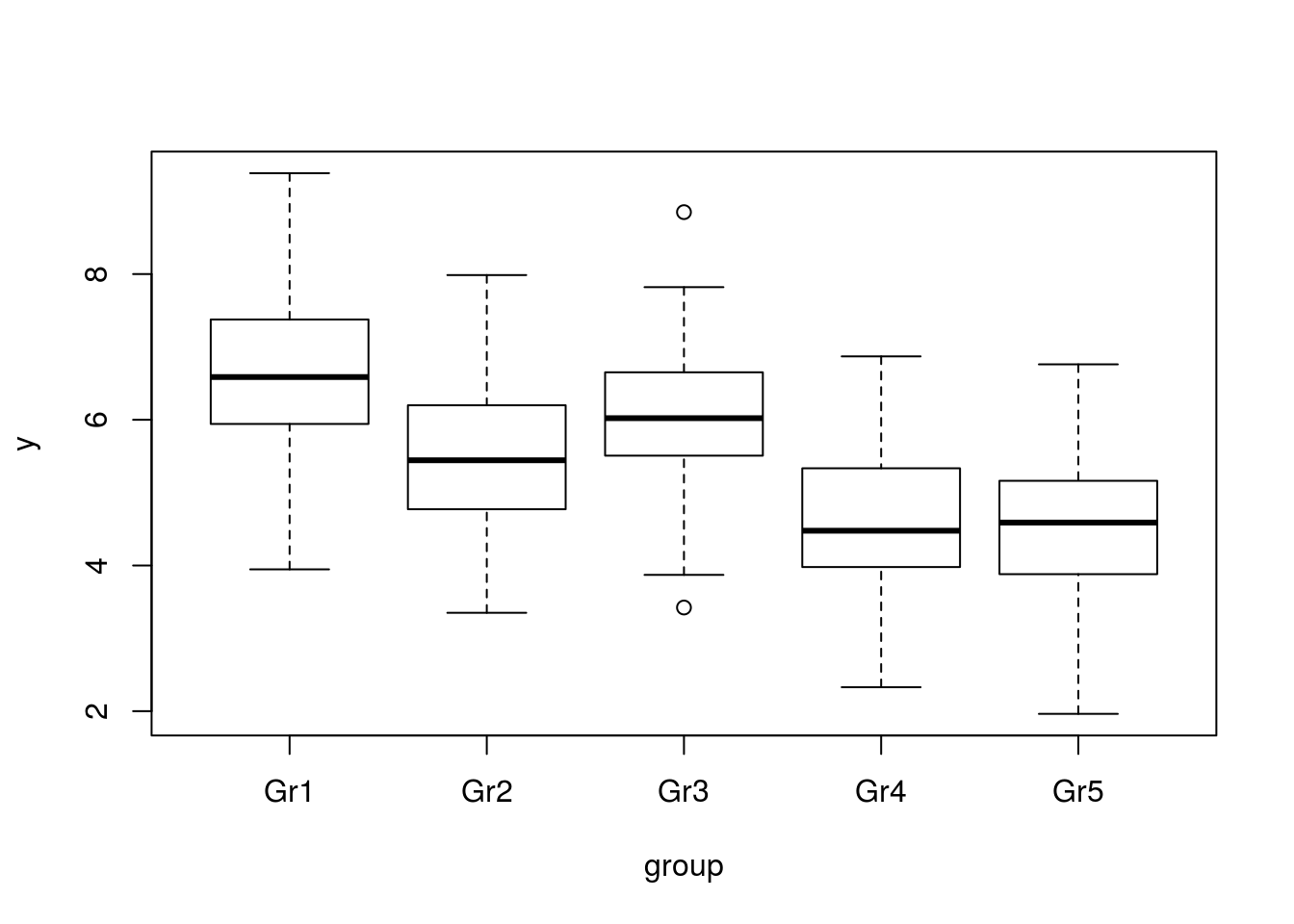stripchart(y ~ group, vertical=TRUE)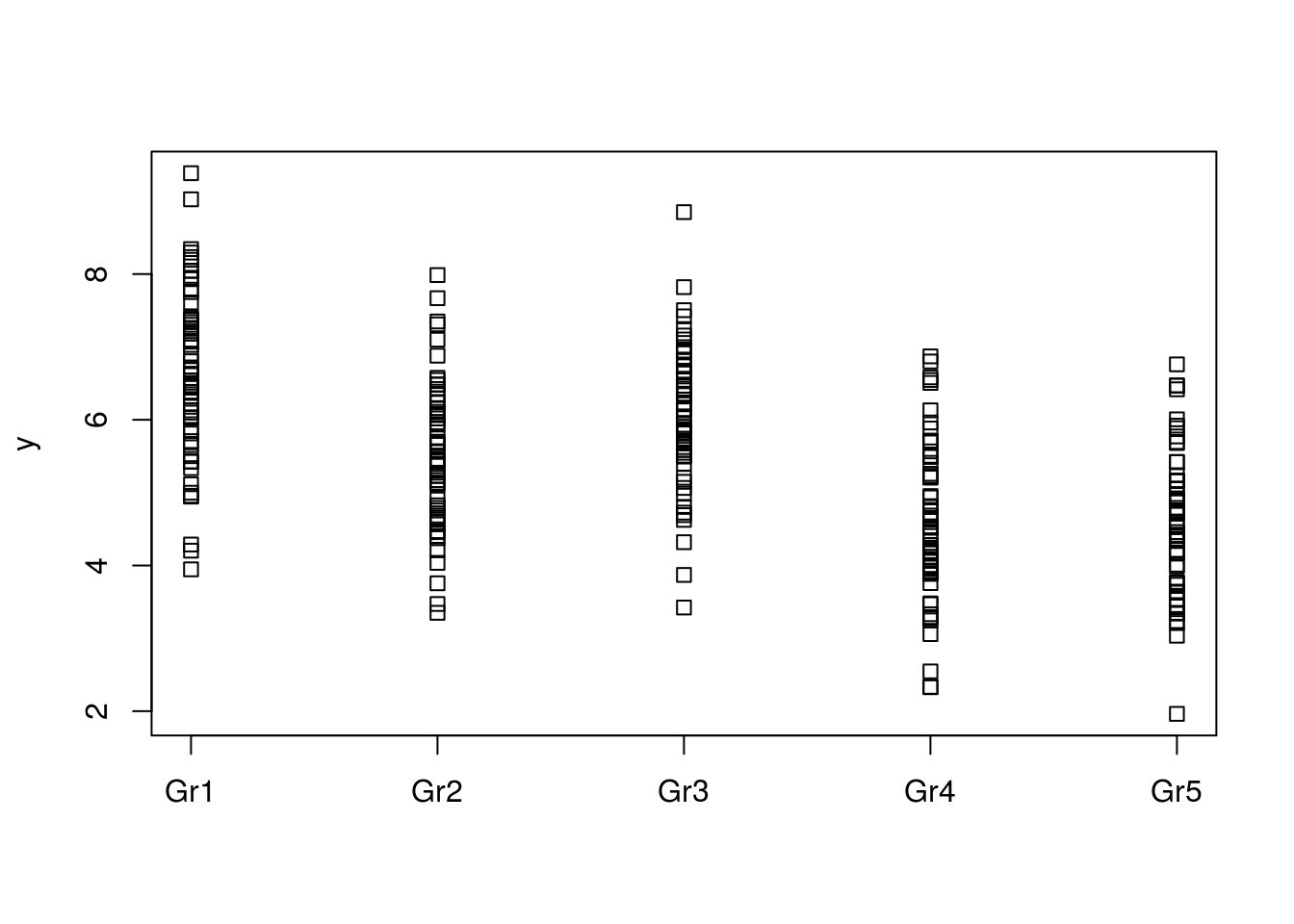for (g in group) { plot(density(y[group==g]), main=g); rug(y[group==g])}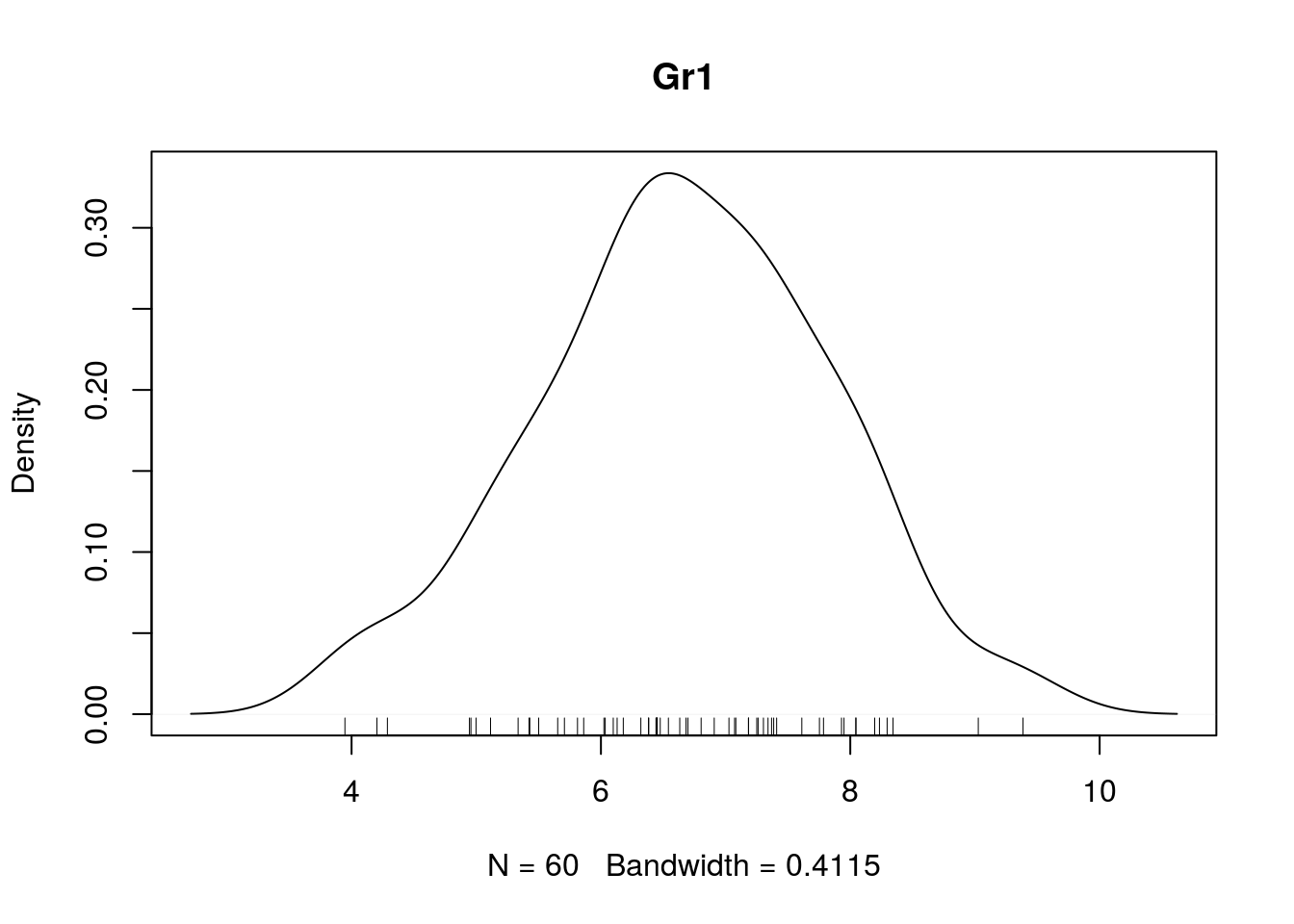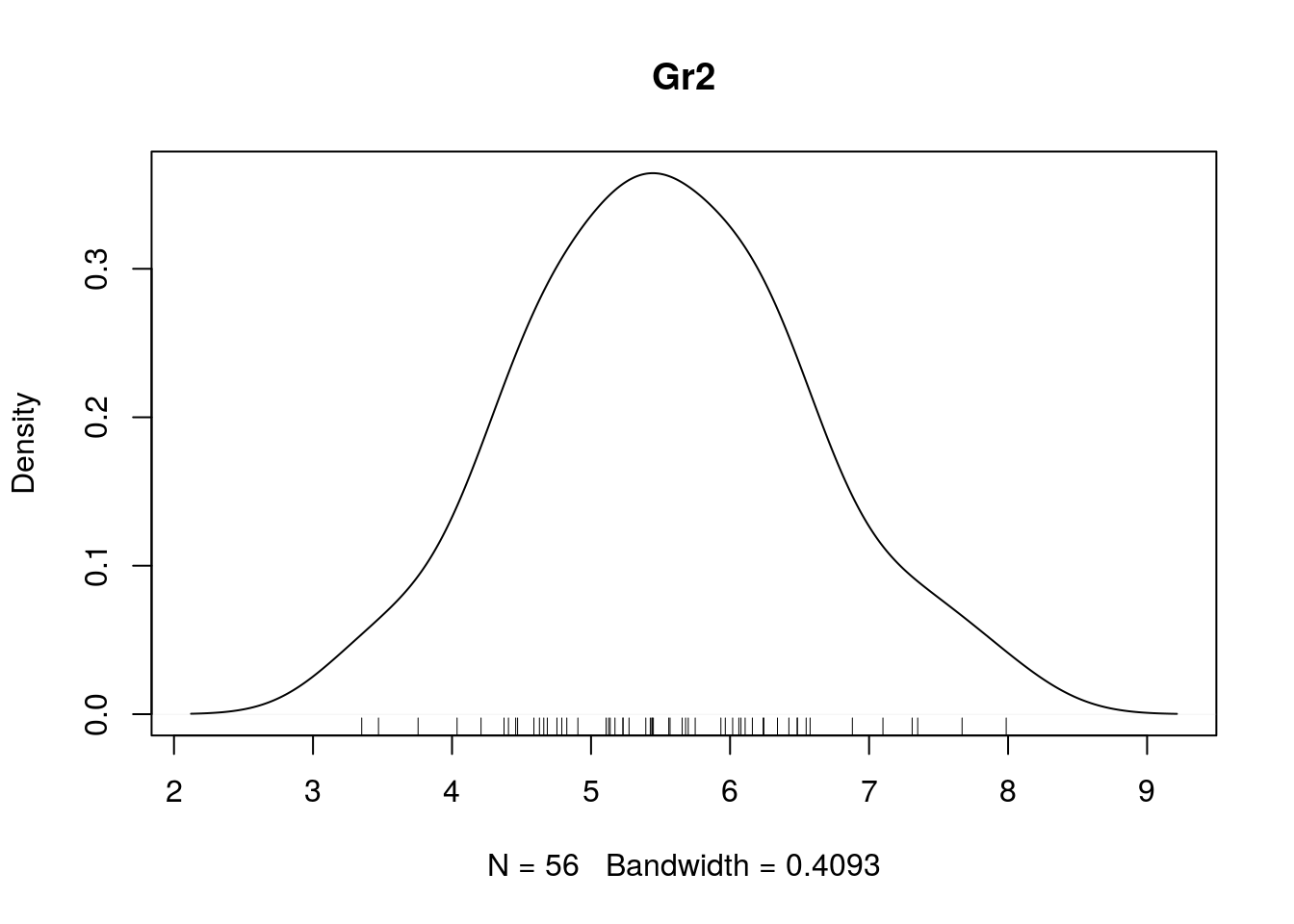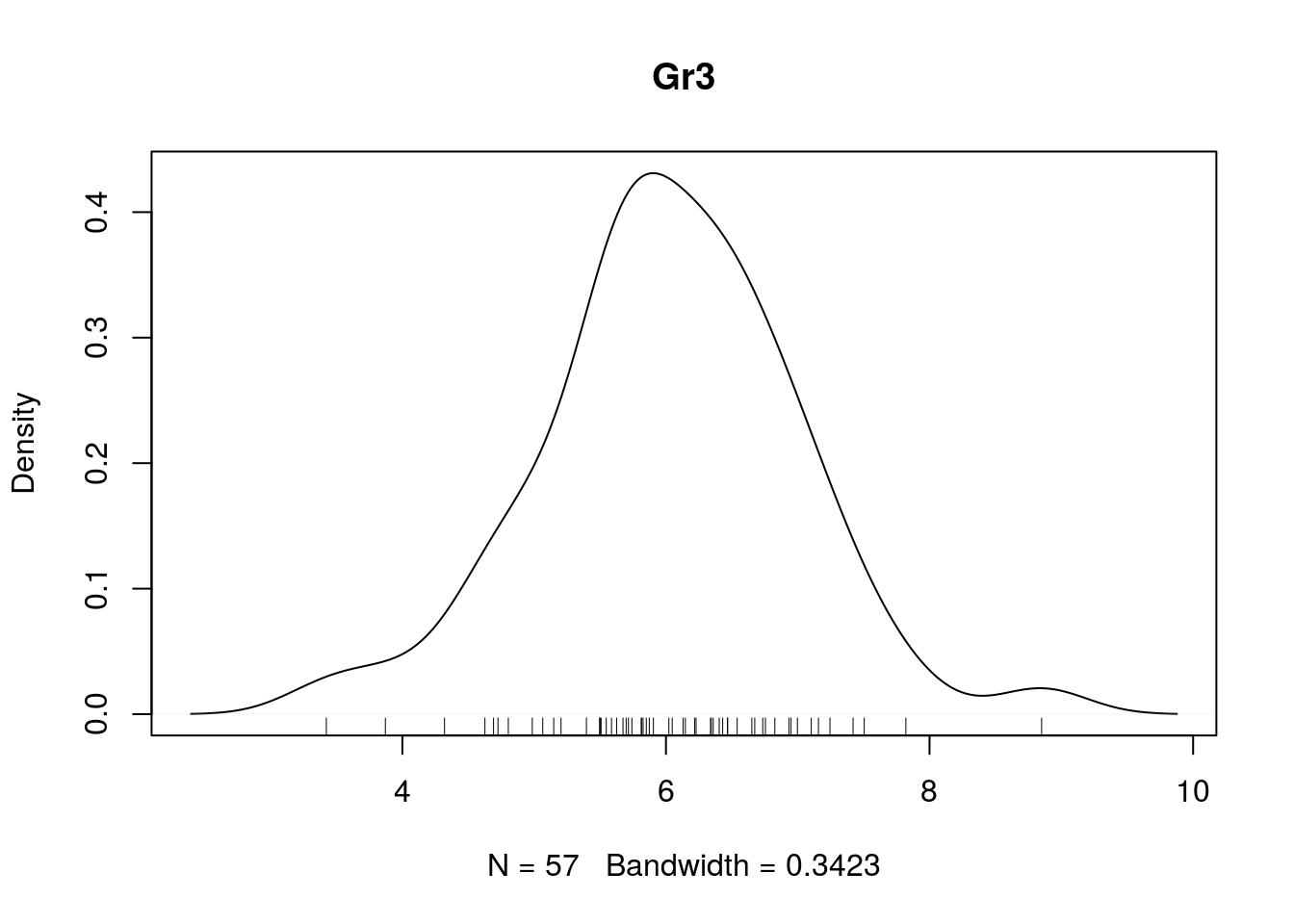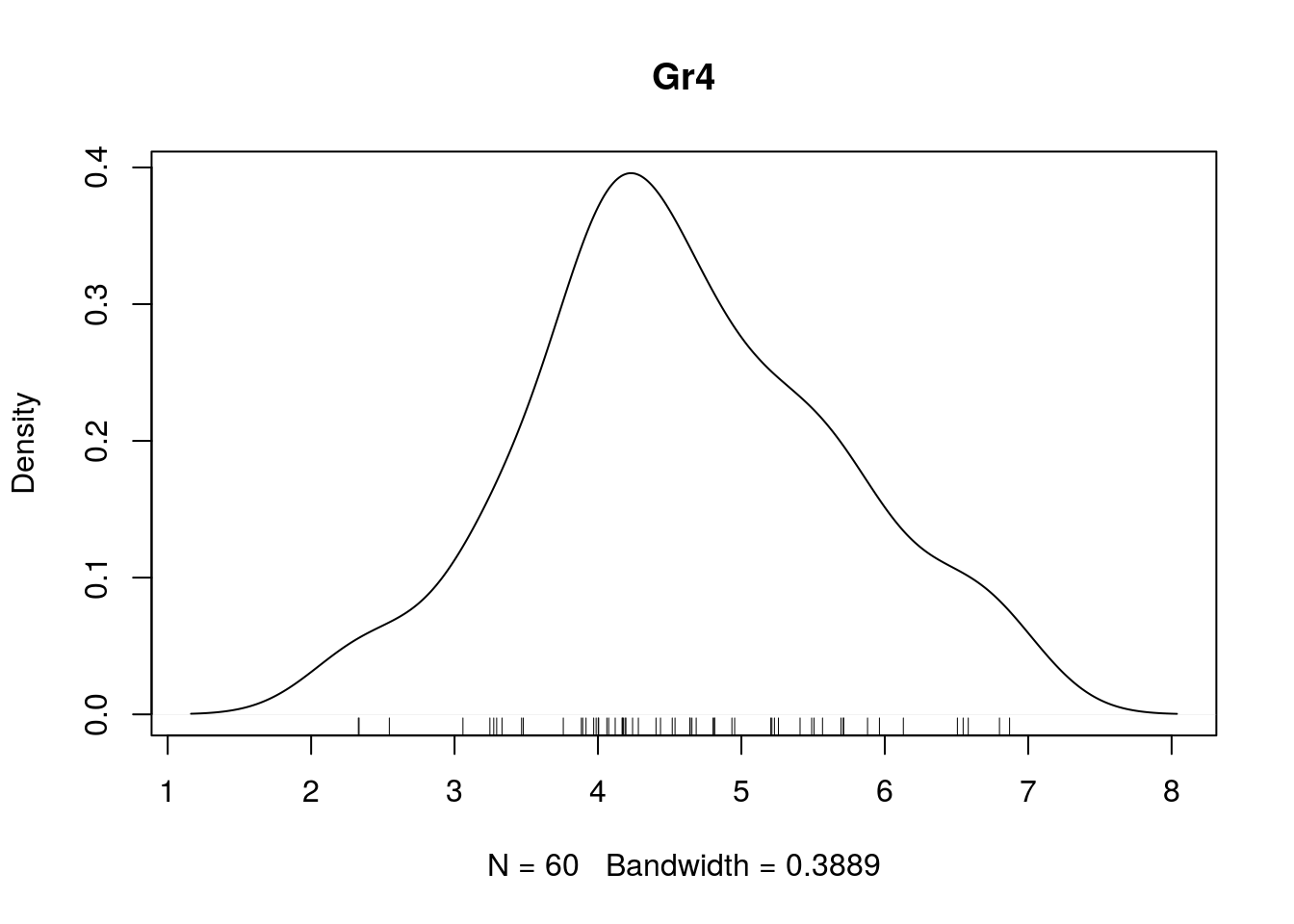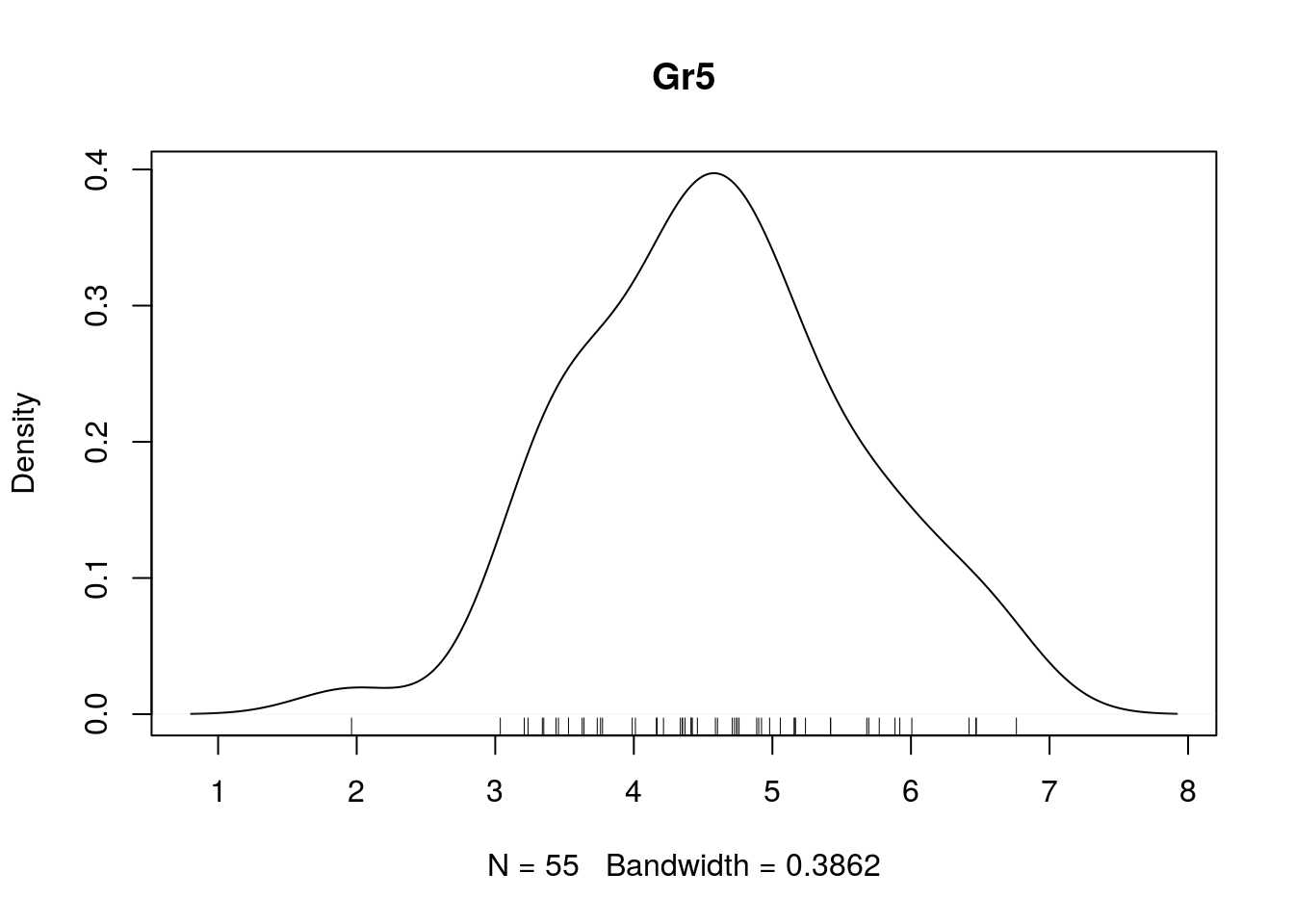detach(ow)

### Descriptive stats

attach(ow)
signif(tapply(y, group, mean),3)
##  Gr1  Gr2  Gr3  Gr4  Gr5
## 6.65 5.53 6.02 4.59 4.60
signif(tapply(y, group, median), 3)
##  Gr1  Gr2  Gr3  Gr4  Gr5
## 6.59 5.44 6.02 4.48 4.59
signif(tapply(y, group, sd), 3)
##   Gr1   Gr2   Gr3   Gr4   Gr5
## 1.160 1.020 0.971 1.070 0.993
signif(tapply(y, group, se), 3)
##   Gr1   Gr2   Gr3   Gr4   Gr5
## 0.150 0.136 0.129 0.139 0.134
detach(ow)

### Inferential statistics

require(ez)
## Loading required package: ez
ow$sub <- factor(1:nrow(ow)) ez_model <- ezANOVA(data=ow, wid=sub, dv=y, between = group) ## Warning: Data is unbalanced (unequal N per group). Make sure you specified ## a well-considered value for the type argument to ezANOVA(). print(ez_model) ##$ANOVA
##   Effect DFn DFd       F            p p<.05       ges
## 1  group   4 283 43.0577 3.347148e-28     * 0.3783373
##
## $Levene's Test for Homogeneity of Variance ## DFn DFd SSn SSd F p p<.05 ## 1 4 283 1.101014 117.1381 0.6649988 0.6167767 ow$sub <- factor(1:nrow(ow))
ezPlot(data = ow,
dv = y,
wid=sub,
between = group,
x = group)  
## Warning: Data is unbalanced (unequal N per group). Make sure you specified
## a well-considered value for the type argument to ezANOVA().
## Warning in ezStats(data = data, dv = dv, wid = wid, within = within,
## within_full = within_full, : Unbalanced groups. Mean N will be used in
## computation of FLSD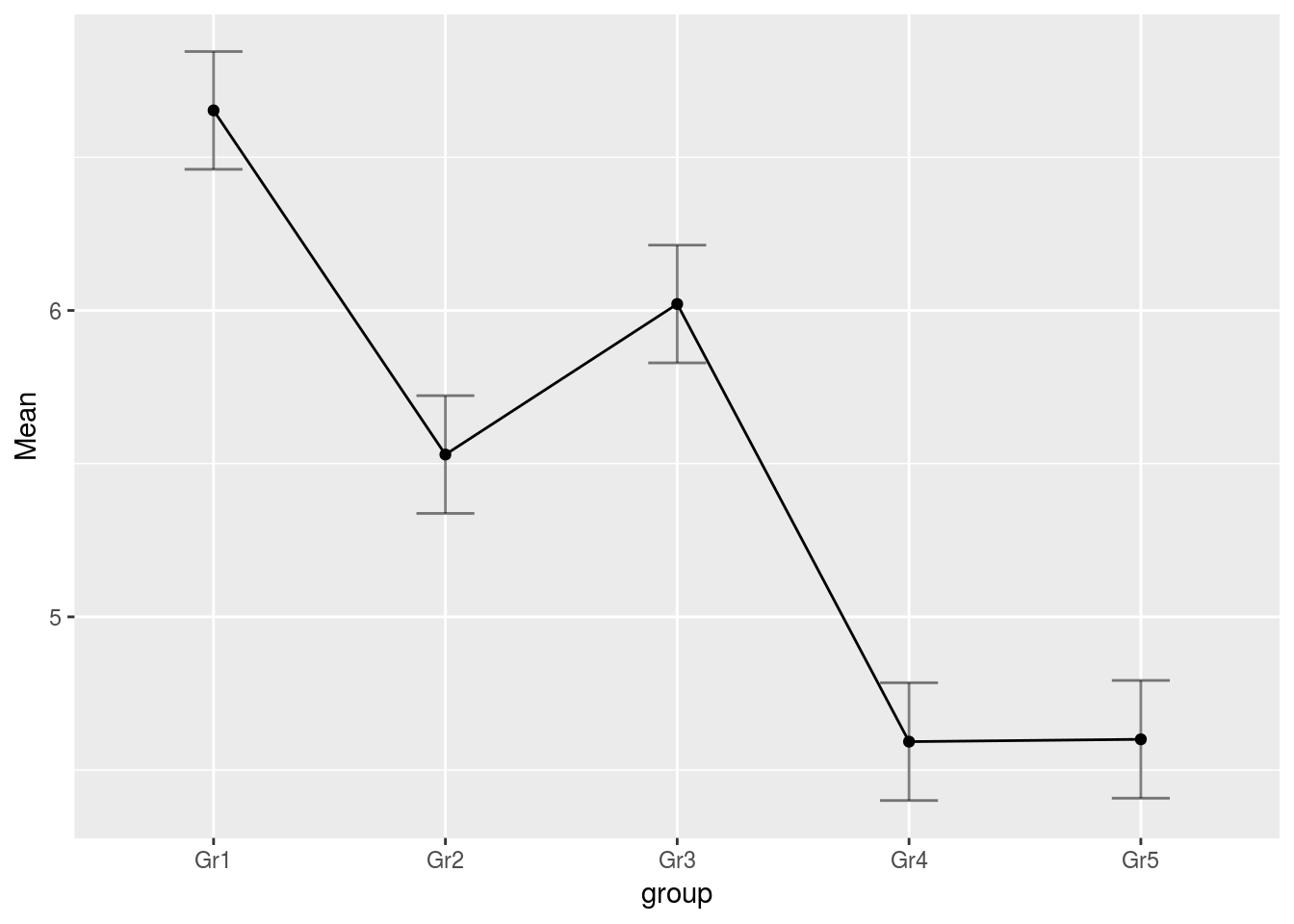summary(av <- aov(y ~ group, data=ow))
##              Df Sum Sq Mean Sq F value Pr(>F)
## group         4  189.1   47.28   43.06 <2e-16 ***
## Residuals   283  310.8    1.10
## ---
## Signif. codes:  0 '***' 0.001 '**' 0.01 '*' 0.05 '.' 0.1 ' ' 1
TukeyHSD(av)
##   Tukey multiple comparisons of means
##     95% family-wise confidence level
##
## Fit: aov(formula = y ~ group, data = ow)
##
## $group ## diff lwr upr p adj ## Gr2-Gr1 -1.123148314 -1.65770790 -0.58858873 0.0000002 ## Gr3-Gr1 -0.631864532 -1.16399318 -0.09973588 0.0108814 ## Gr4-Gr1 -2.060103427 -2.58536561 -1.53484124 0.0000000 ## Gr5-Gr1 -2.052525914 -2.58959322 -1.51545861 0.0000000 ## Gr3-Gr2 0.491283782 -0.05002434 1.03259190 0.0953760 ## Gr4-Gr2 -0.936955114 -1.47151470 -0.40239553 0.0000239 ## Gr5-Gr2 -0.929377600 -1.47554138 -0.38321382 0.0000452 ## Gr4-Gr3 -1.428238896 -1.96036754 -0.89611025 0.0000000 ## Gr5-Gr3 -1.420661382 -1.96444610 -0.87687666 0.0000000 ## Gr5-Gr4 0.007577513 -0.52948979 0.54464482 0.9999995 plot(TukeyHSD(av))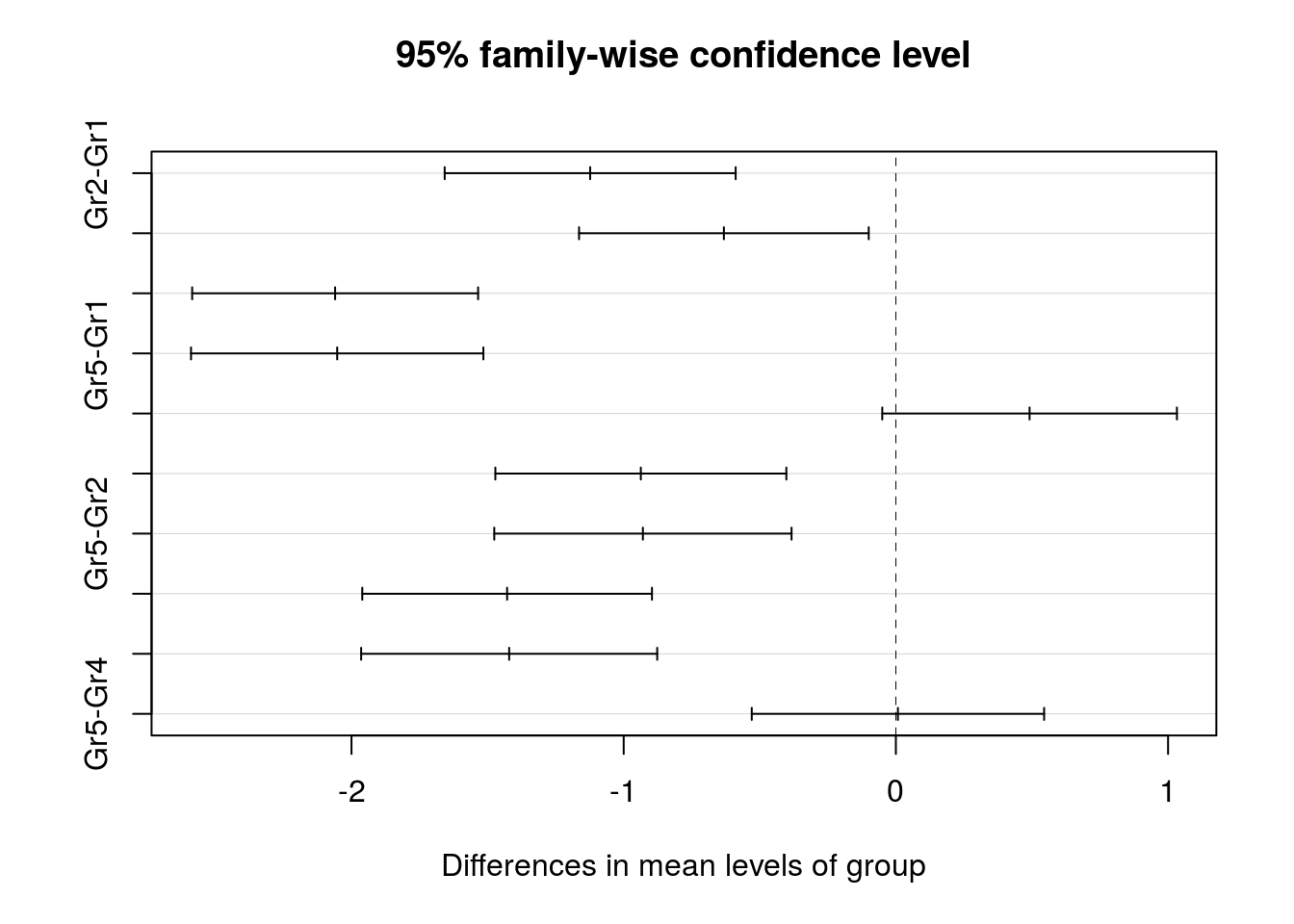The output of lm provides additonal information contrasts(ow$group) <- contr.treatment
summary(lmtr <- lm(y ~ group, data=ow))
##
## Call:
## lm(formula = y ~ group, data = ow)
##
## Residuals:
##      Min       1Q   Median       3Q      Max
## -2.70612 -0.62439 -0.07799  0.65597  2.82940
##
## Coefficients:
##             Estimate Std. Error t value Pr(>|t|)
## (Intercept)   6.6528     0.1353  49.177  < 2e-16 ***
## group2       -1.1231     0.1947  -5.768  2.1e-08 ***
## group3       -0.6319     0.1938  -3.260  0.00125 **
## group4       -2.0601     0.1913 -10.768  < 2e-16 ***
## group5       -2.0525     0.1956 -10.492  < 2e-16 ***
## ---
## Signif. codes:  0 '***' 0.001 '**' 0.01 '*' 0.05 '.' 0.1 ' ' 1
##
## Residual standard error: 1.048 on 283 degrees of freedom
## Multiple R-squared:  0.3783, Adjusted R-squared:  0.3696
## F-statistic: 43.06 on 4 and 283 DF,  p-value: < 2.2e-16
contrasts(ow$group) <- contr.sum summary(lmsum <- lm(y ~ group, data=ow)) ## ## Call: ## lm(formula = y ~ group, data = ow) ## ## Residuals: ## Min 1Q Median 3Q Max ## -2.70612 -0.62439 -0.07799 0.65597 2.82940 ## ## Coefficients: ## Estimate Std. Error t value Pr(>|t|) ## (Intercept) 5.47931 0.06179 88.679 < 2e-16 *** ## group1 1.17353 0.12165 9.647 < 2e-16 *** ## group2 0.05038 0.12483 0.404 0.687 ## group3 0.54166 0.12400 4.368 1.76e-05 *** ## group4 -0.88657 0.12165 -7.288 3.15e-12 *** ## --- ## Signif. codes: 0 '***' 0.001 '**' 0.01 '*' 0.05 '.' 0.1 ' ' 1 ## ## Residual standard error: 1.048 on 283 degrees of freedom ## Multiple R-squared: 0.3783, Adjusted R-squared: 0.3696 ## F-statistic: 43.06 on 4 and 283 DF, p-value: < 2.2e-16 # Comparing two treatments In the previous section, the data from the two groups were assumed to be independent. If there is some pairing, for example if data were acquired in the same unit under two conditions, then the data are not independent. The simplest way to perform the data analysis is to examine the differences between the two conditions computed over each unit. Here data come organized a long table format with one measure per row, and condition and subject as variables. This less convenient to compute the differences within subjects than a short format with one subject per row, and one column per condition, but better to run linear model. To convert from one representation to the other, see stack, reshape2, plyr… tc <- read.csv("twotreat.csv") head(tc) ## X sub cond y ## 1 1 s1 1 9.391306 ## 2 2 s1 2 10.928504 ## 3 3 s2 1 8.133601 ## 4 4 s2 2 10.383381 ## 5 5 s3 1 10.356377 ## 6 6 s3 2 11.435402 str(tc) ## 'data.frame': 40 obs. of 4 variables: ##$ X   : int  1 2 3 4 5 6 7 8 9 10 ...
##  $sub : Factor w/ 20 levels "s1","s10","s11",..: 1 1 12 12 14 14 15 15 16 16 ... ##$ cond: int  1 2 1 2 1 2 1 2 1 2 ...
##  $y : num 9.39 10.93 8.13 10.38 10.36 ... tc$sub <- factor(tc$sub) # make sure these vars are factors tc$cond <- factor(tc$cond) table(tc$sub)
##
##  s1 s10 s11 s12 s13 s14 s15 s16 s17 s18 s19  s2 s20  s3  s4  s5  s6  s7
##   2   2   2   2   2   2   2   2   2   2   2   2   2   2   2   2   2   2
##  s8  s9
##   2   2

(I assume that there are no repeated measures within subject and treatment. If this is the case with your dataset, use aggregate or melt)

### Graphical explorations

with(tc, interaction.plot(cond, sub, y))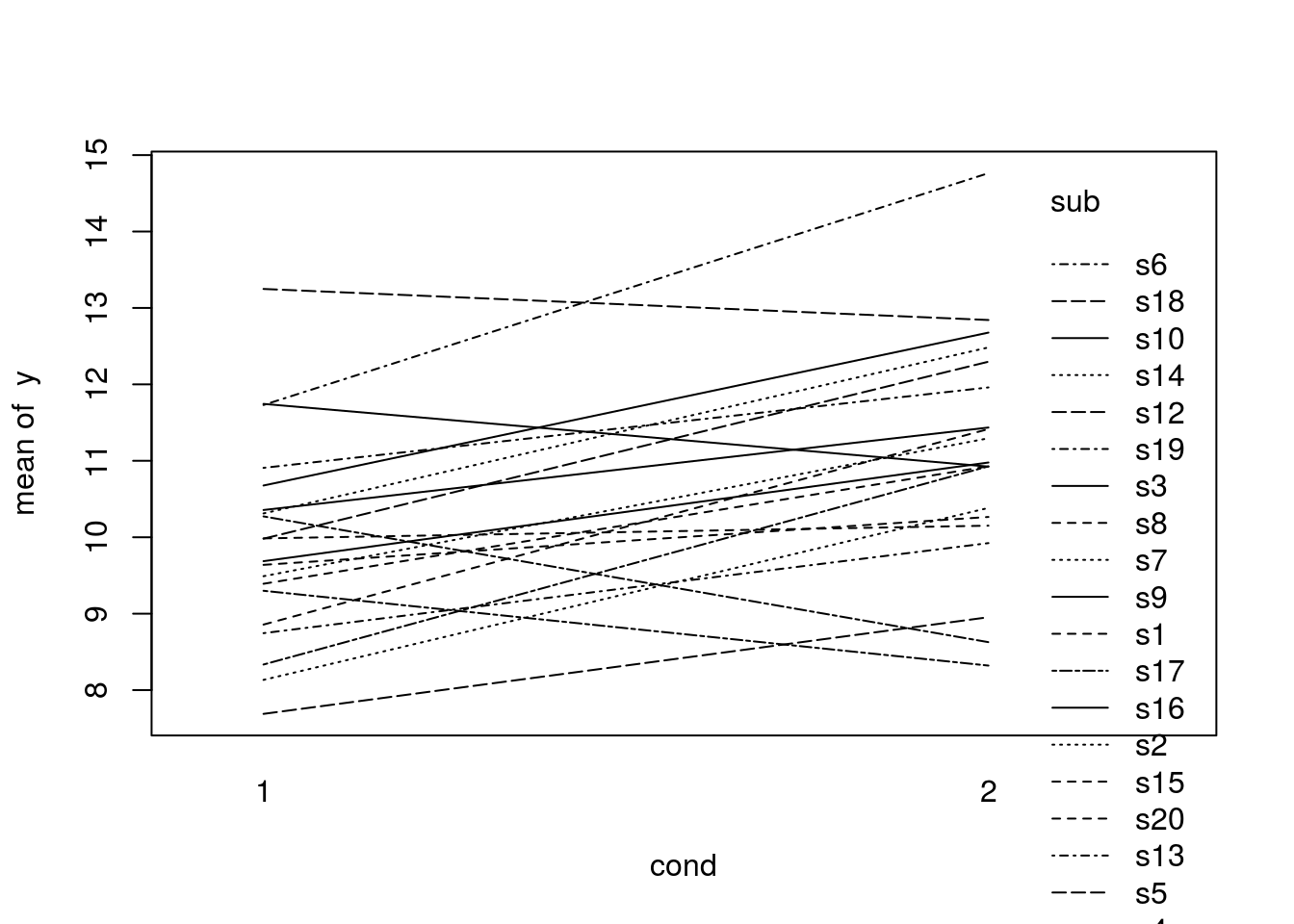Fancier graphs can be obtained with lattice:

require(lattice)
xyplot(y ~ cond, group=sub, data=tc, type='l')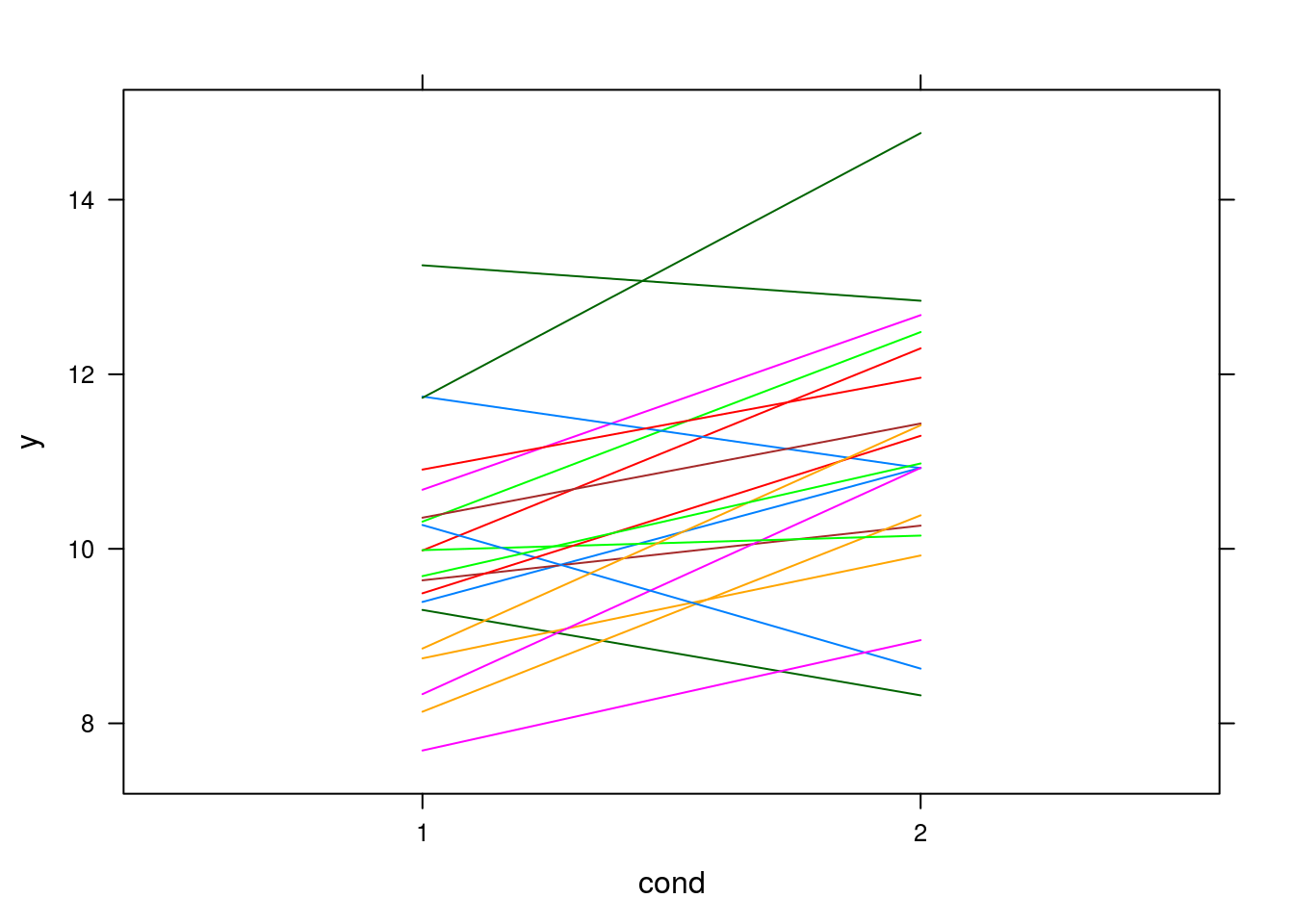xyplot(y ~ cond | sub, data=tc, type='l')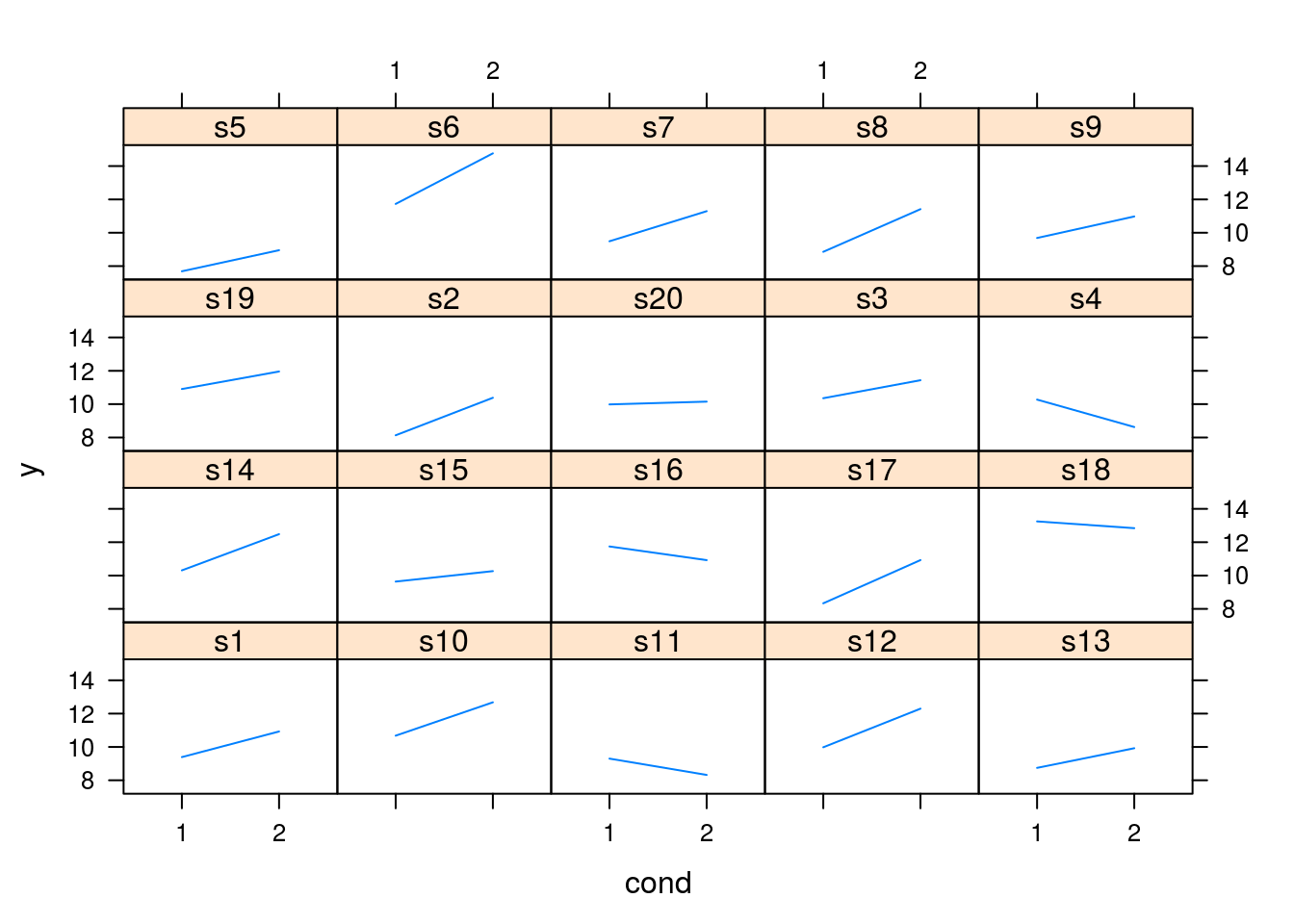We can also remove to main effects of subjects, as we are interested in the difference between condition within subjects:

attach(tc)
c2 <- levels(tc$cond) s1 <- tc$sub[tc$cond==c1] y1 <- tc$y[tc$cond==c1][order(s1)] s2 <- tc$sub[tc$cond==c2] y2 <- tc$y[tc$cond==c2][order(s2)] summary(y1-y2) ## Min. 1st Qu. Median Mean 3rd Qu. Max. ## -3.0330 -2.1910 -1.2780 -1.1540 -0.5116 1.6450 se(y1-y2) # standard error of the effect ##  0.2922856 # Check if the pairing was useful? cor.test(y1, y2) ## ## Pearson's product-moment correlation ## ## data: y1 and y2 ## t = 3.1518, df = 18, p-value = 0.005517 ## alternative hypothesis: true correlation is not equal to 0 ## 95 percent confidence interval: ## 0.2089560 0.8219508 ## sample estimates: ## cor ## 0.5963352 ### Inferential stats t.test(y1, y2, paired=T) ## ## Paired t-test ## ## data: y1 and y2 ## t = -3.9467, df = 19, p-value = 0.0008653 ## alternative hypothesis: true difference in means is not equal to 0 ## 95 percent confidence interval: ## -1.7653345 -0.5418128 ## sample estimates: ## mean of the differences ## -1.153574 Linear model approach (sm <- summary(model_lm <- lm(y ~ cond + sub, data=tc))) ## ## Call: ## lm(formula = y ~ cond + sub, data = tc) ## ## Residuals: ## Min 1Q Median 3Q Max ## -1.3995 -0.4974 0.0000 0.4974 1.3995 ## ## Coefficients: ## Estimate Std. Error t value Pr(>|t|) ## (Intercept) 9.58312 0.66971 14.309 1.26e-11 *** ## cond2 1.15357 0.29229 3.947 0.000865 *** ## subs10 1.51722 0.92429 1.641 0.117144 ## subs11 -1.34969 0.92429 -1.460 0.160561 ## subs12 0.97900 0.92429 1.059 0.302790 ## subs13 -0.82581 0.92429 -0.893 0.382791 ## subs14 1.23746 0.92429 1.339 0.196428 ## subs15 -0.20772 0.92429 -0.225 0.824583 ## subs16 1.17449 0.92429 1.271 0.219177 ## subs17 -0.52878 0.92429 -0.572 0.573968 ## subs18 2.88544 0.92429 3.122 0.005615 ** ## subs19 1.27412 0.92429 1.378 0.184068 ## subs2 -0.90141 0.92429 -0.975 0.341689 ## subs20 -0.09180 0.92429 -0.099 0.921921 ## subs3 0.73598 0.92429 0.796 0.435711 ## subs4 -0.70989 0.92429 -0.768 0.451905 ## subs5 -1.83862 0.92429 -1.989 0.061269 . ## subs6 3.08695 0.92429 3.340 0.003442 ** ## subs7 0.23268 0.92429 0.252 0.803946 ## subs8 -0.02338 0.92429 -0.025 0.980080 ## subs9 0.17251 0.92429 0.187 0.853918 ## --- ## Signif. codes: 0 '***' 0.001 '**' 0.01 '*' 0.05 '.' 0.1 ' ' 1 ## ## Residual standard error: 0.9243 on 19 degrees of freedom ## Multiple R-squared: 0.8245, Adjusted R-squared: 0.6398 ## F-statistic: 4.464 on 20 and 19 DF, p-value: 0.0009515 (diff <-sm$coefficients[2,'Estimate'])
##  1.153574
(diffse <- sm$coefficients[2,'Std. Error']) ##  0.2922856 In this simple situation, mixed effect models will yield the same p-values: require(nlme) ## Loading required package: nlme (model_lme <- lme(y ~ cond, random=~1|sub, data= tc)) ## Linear mixed-effects model fit by REML ## Data: tc ## Log-restricted-likelihood: -66.80189 ## Fixed: y ~ cond ## (Intercept) cond2 ## 9.924055 1.153574 ## ## Random effects: ## Formula: ~1 | sub ## (Intercept) Residual ## StdDev: 1.108976 0.9242883 ## ## Number of Observations: 40 ## Number of Groups: 20 summary(model_lme) ## Linear mixed-effects model fit by REML ## Data: tc ## AIC BIC logLik ## 141.6038 148.1541 -66.80189 ## ## Random effects: ## Formula: ~1 | sub ## (Intercept) Residual ## StdDev: 1.108976 0.9242883 ## ## Fixed effects: y ~ cond ## Value Std.Error DF t-value p-value ## (Intercept) 9.924055 0.3228109 19 30.742626 0e+00 ## cond2 1.153574 0.2922856 19 3.946734 9e-04 ## Correlation: ## (Intr) ## cond2 -0.453 ## ## Standardized Within-Group Residuals: ## Min Q1 Med Q3 Max ## -1.80719196 -0.44122630 -0.04697454 0.44392016 1.78263759 ## ## Number of Observations: 40 ## Number of Groups: 20 # plot(ranef(model_lme)) # plot(res_lme <- residuals(model_lme)) # qqnorm(res_lme) # qqline(res_lme) # plot(model_lme) require(lme4) ## Loading required package: lme4 ## Loading required package: Matrix ## ## Attaching package: 'lme4' ## The following object is masked from 'package:nlme': ## ## lmList (model_lmer <- lmer(y ~ cond + (1|sub), data= tc)) ## Linear mixed model fit by REML ['lmerMod'] ## Formula: y ~ cond + (1 | sub) ## Data: tc ## REML criterion at convergence: 133.6038 ## Random effects: ## Groups Name Std.Dev. ## sub (Intercept) 1.1090 ## Residual 0.9243 ## Number of obs: 40, groups: sub, 20 ## Fixed Effects: ## (Intercept) cond2 ## 9.924 1.154 summary(model_lmer) ## Linear mixed model fit by REML ['lmerMod'] ## Formula: y ~ cond + (1 | sub) ## Data: tc ## ## REML criterion at convergence: 133.6 ## ## Scaled residuals: ## Min 1Q Median 3Q Max ## -1.80719 -0.44123 -0.04697 0.44392 1.78264 ## ## Random effects: ## Groups Name Variance Std.Dev. ## sub (Intercept) 1.2298 1.1090 ## Residual 0.8543 0.9243 ## Number of obs: 40, groups: sub, 20 ## ## Fixed effects: ## Estimate Std. Error t value ## (Intercept) 9.9241 0.3228 30.743 ## cond2 1.1536 0.2923 3.947 ## ## Correlation of Fixed Effects: ## (Intr) ## cond2 -0.453 # qqmath(ranef(model_lmer)) Bootstrap confidence interval for the difference require(boot) samplemean <- function(x, d) { mean(x[d]) } b <- boot(y1-y2, samplemean, 1000) boot.ci(b) ## Warning in boot.ci(b): bootstrap variances needed for studentized intervals ## BOOTSTRAP CONFIDENCE INTERVAL CALCULATIONS ## Based on 1000 bootstrap replicates ## ## CALL : ## boot.ci(boot.out = b) ## ## Intervals : ## Level Normal Basic ## 95% (-1.705, -0.603 ) (-1.734, -0.641 ) ## ## Level Percentile BCa ## 95% (-1.666, -0.574 ) (-1.630, -0.498 ) ## Calculations and Intervals on Original Scale ### Plots The errors bars can either represent the standard errors (or confidence intervals) of the means of each treatment, or the standard error bar for the difference between the two treatments when intersubject variability is taken out. First graphics: with the std.err. of the means: attach(tc) par(mfrow=c(1,1)) means <- tapply(y, cond, mean) (ses <- tapply(y, cond, se)) ## 1 2 ## 0.2986051 0.3453241 ysca = c(min(means-3*ses), max(means+3*ses)) mp <- barplot(means, ylim=ysca, xpd=F) arrows(mp, means-ses, mp, means+ses, code=3, angle=90)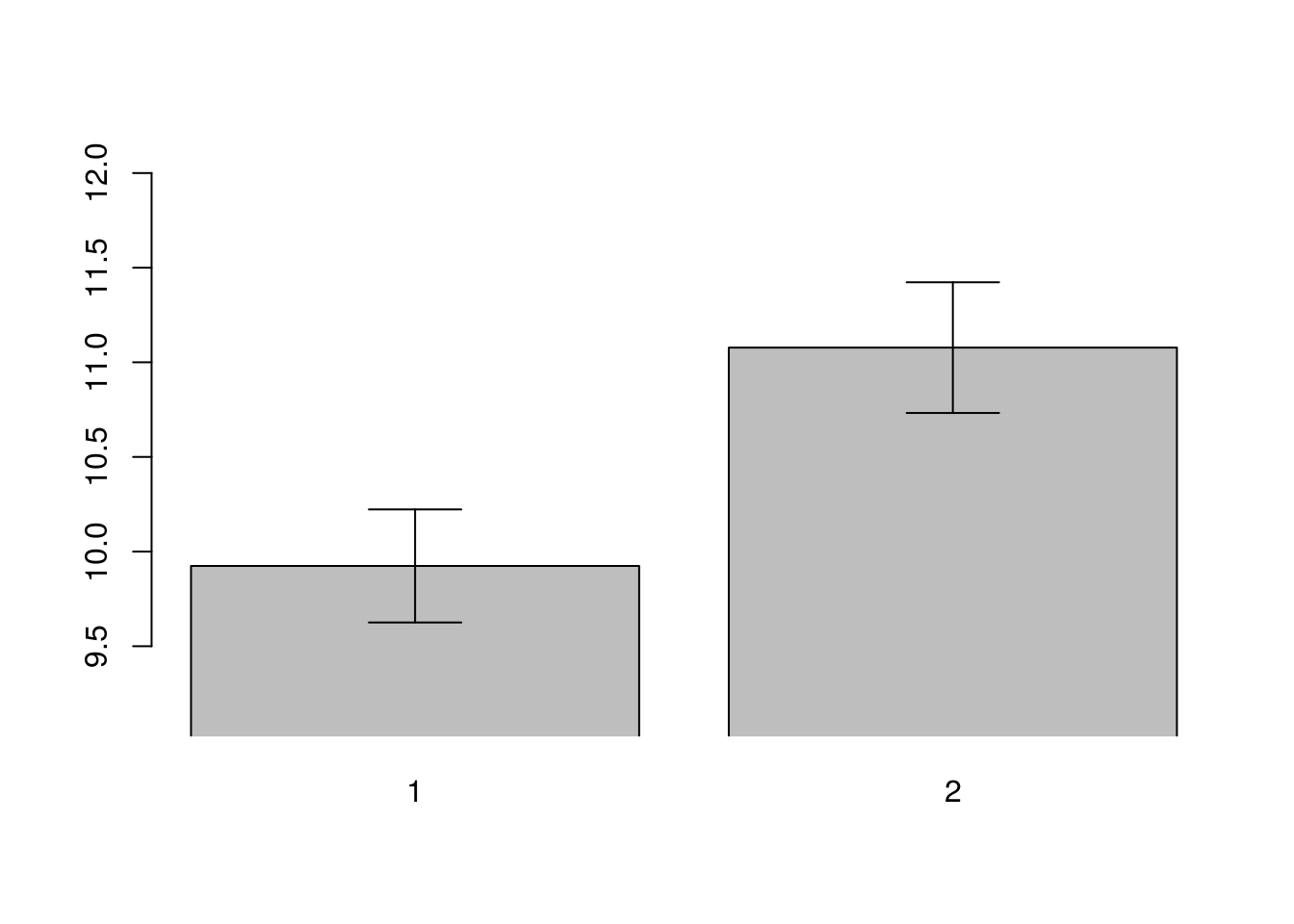detach(tc) If we remove the between Ss variability attach(tc) par(mfrow=c(1,1)) means <- tapply(y, cond, mean) (ses <- tapply(ycorr, cond, se)) ## 1 2 ## 0.1461428 0.1461428 ysca = c(min(means-3*ses), max(means+3*ses)) mp <- barplot(means, ylim=ysca, xpd=F) arrows(mp, means-ses, mp, means+ses, code=3, angle=90)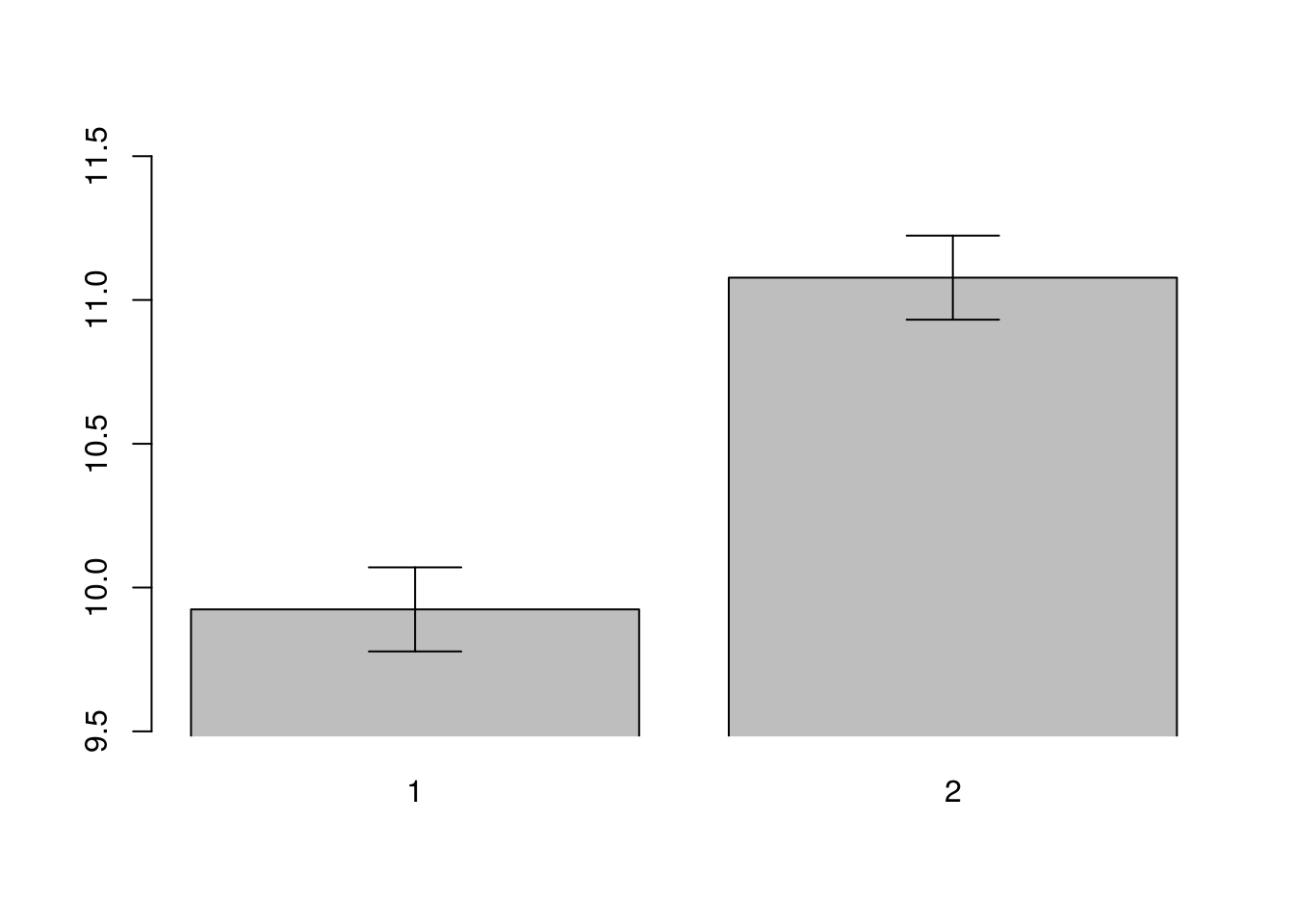detach(tc) If we take the standard error from the regression: (sm <- summary(model_lm <- lm(y ~ cond + sub, data=tc))) ## ## Call: ## lm(formula = y ~ cond + sub, data = tc) ## ## Residuals: ## Min 1Q Median 3Q Max ## -1.3995 -0.4974 0.0000 0.4974 1.3995 ## ## Coefficients: ## Estimate Std. Error t value Pr(>|t|) ## (Intercept) 9.58312 0.66971 14.309 1.26e-11 *** ## cond2 1.15357 0.29229 3.947 0.000865 *** ## subs10 1.51722 0.92429 1.641 0.117144 ## subs11 -1.34969 0.92429 -1.460 0.160561 ## subs12 0.97900 0.92429 1.059 0.302790 ## subs13 -0.82581 0.92429 -0.893 0.382791 ## subs14 1.23746 0.92429 1.339 0.196428 ## subs15 -0.20772 0.92429 -0.225 0.824583 ## subs16 1.17449 0.92429 1.271 0.219177 ## subs17 -0.52878 0.92429 -0.572 0.573968 ## subs18 2.88544 0.92429 3.122 0.005615 ** ## subs19 1.27412 0.92429 1.378 0.184068 ## subs2 -0.90141 0.92429 -0.975 0.341689 ## subs20 -0.09180 0.92429 -0.099 0.921921 ## subs3 0.73598 0.92429 0.796 0.435711 ## subs4 -0.70989 0.92429 -0.768 0.451905 ## subs5 -1.83862 0.92429 -1.989 0.061269 . ## subs6 3.08695 0.92429 3.340 0.003442 ** ## subs7 0.23268 0.92429 0.252 0.803946 ## subs8 -0.02338 0.92429 -0.025 0.980080 ## subs9 0.17251 0.92429 0.187 0.853918 ## --- ## Signif. codes: 0 '***' 0.001 '**' 0.01 '*' 0.05 '.' 0.1 ' ' 1 ## ## Residual standard error: 0.9243 on 19 degrees of freedom ## Multiple R-squared: 0.8245, Adjusted R-squared: 0.6398 ## F-statistic: 4.464 on 20 and 19 DF, p-value: 0.0009515 diff <-sm$coefficients[2,'Estimate']
diffse <- sm\$coefficients[2,'Std. Error']

attach(tc)
par(mfrow=c(1,1))

means <- tapply(y, cond, mean)
(ses <- rep(diffse, length(means)))
##  0.2922856 0.2922856
ysca = c(min(means-3*ses), max(means+3*ses))

mp <- barplot(means, ylim=ysca, xpd=F)
arrows(mp, means-ses,
mp, means+ses,
code=3, angle=90)